# MachineShop User Guide

### R Package Version 3.6.2

University of Iowa
brian-j-smith@uiowa.edu

# 1 Description

MachineShop is a meta-package for statistical and machine learning with a unified interface for model fitting, prediction, performance assessment, and presentation of results. Support is provided for predictive modeling of numerical, categorical, and censored time-to-event outcomes and for resample (bootstrap, cross-validation, and split training-test sets) estimation of model performance. This vignette introduces the package interface with a survival data analysis example, followed by supported methods of variable specification; applications to other response variable types; available performance metrics, resampling techniques, and graphical and tabular summaries; and modeling strategies.

# 2 Features

• Unified and concise interface for model fitting, prediction, and performance assessment.
• Support for 53+ models from 28 R packages, including model specifications from the parsnip package.
• Dynamic model parameters.
• Ensemble modeling with stacked regression and super learners.
• Modeling of response variables types: binary factors, multi-class nominal and ordinal factors, numeric vectors and matrices, and censored time-to-event survival.
• Model specification with traditional formulas, design matrices, and flexible pre-processing recipes.
• Resample estimation of predictive performance, including cross-validation, bootstrap resampling, and split training-test set validation.
• Parallel execution of resampling algorithms.
• Choices of performance metrics: accuracy, areas under ROC and precision recall curves, Brier score, coefficient of determination (R2), concordance index, cross entropy, F score, Gini coefficient, unweighted and weighted Cohen’s kappa, mean absolute error, mean squared error, mean squared log error, positive and negative predictive values, precision and recall, and sensitivity and specificity.
• Graphical and tabular performance summaries: calibration curves, confusion matrices, partial dependence plots, performance curves, lift curves, and model-specific and permutation-based variable importance.
• Model tuning over automatically generated grids and with exhaustive and random grid searches, Bayesian optimization, particle swarm optimization, quasi-Newton BFGS optimization, simulated annealing, and support for user-defined optimization functions.
• Model selection and comparisons for any combination of models and model parameter values.
• Recursive feature elimination.
• User-definable models and performance metrics.

# 3 Getting Started

## 3.1 Installation

# Current release from CRAN
install.packages("MachineShop")

# Development version from GitHub
# install.packages("devtools")
devtools::install_github("brian-j-smith/MachineShop")

# Development version with vignettes
devtools::install_github("brian-j-smith/MachineShop", build_vignettes = TRUE)

## 3.2 Documentation

Once installed, the following R commands will load the package and display its help system documentation. Online documentation and examples are available at the MachineShop website.

library(MachineShop)

# Package help summary
?MachineShop

# Vignette
RShowDoc("UserGuide", package = "MachineShop")

# 4 Melanoma Example

Use of the MachineShop package is demonstrated with a survival analysis example in which the response variable is a censored time to event outcome. Since survival outcomes are a combination of numerical (time to event) and categorical (event) variables, package features for both variable types are illustrated with the example. Support for outcomes other than survival, including nominal and ordinal factors as well as numeric vectors and matrices, is also discussed.

Survival analysis is performed with the Melanoma dataset from the MASS package (Andersen et al. 1993). This dataset provides survival time, in days, from disease treatment to (1) death from disease, (2) alive at study termination, or (3) death from other causes for 205 Denmark patients with malignant melanomas. Also provided are potential predictors of the survival outcomes. The analysis begins by loading required packages MachineShop, survival (Therneau 2020), and MASS. For the analysis, a binary overall survival outcome is created by combining the two death categories (1 and 3) into one.

## Analysis libraries and dataset
library(MachineShop)
library(survival)
data(Melanoma, package = "MASS")

## Malignant melanoma analysis dataset
surv_df <- within(Melanoma, {
y <- Surv(time, status != 2)
remove(time, status)
})

Descriptive summaries of the study variables are given below in Table 1, followed by a plot of estimated overall survival probabilities and 95% confidence intervals.

Table 1. Variable summaries for the Melanoma survival analysis example.
Characteristic Value
Number of subjects 205
time
Median (Range) 2005 (10, 5565)
status
0 = Alive 134 (65.37%)
sex
1 = Male 79 (38.54%)
0 = Female 126 (61.46%)
age
Median (Range) 54 (4, 95)
year
Median (Range) 1970 (1962, 1977)
thickness
Median (Range) 1.94 (0.10, 17.42)
ulcer
1 = Presence 90 (43.9%)
0 = Absence 115 (56.1%)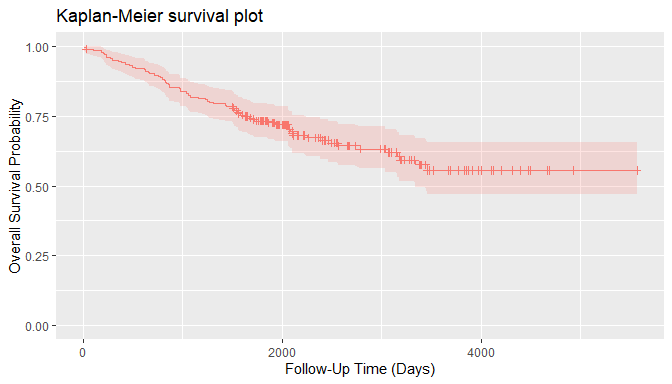For the analyses, the dataset is split into a training set on which survival models will be fit and a test set on which predictions will be made and performance evaluated. A global formula surv_fo is defined to relate the predictors on the right hand side to the overall survival outcome on the left and will be used for all subsequent survival models.

## Training and test sets
set.seed(123)
train_indices <- sample(nrow(surv_df), nrow(surv_df) * 2 / 3)
surv_train <- surv_df[train_indices, ]
surv_test <- surv_df[-train_indices, ]

## Global formula for the analysis
surv_fo <- y ~ sex + age + year + thickness + ulcer

# 5 Model Fit and Prediction

## 5.1 Model Information

Model fitting requires user specification of a MachineShop compatible model. A named list of package-supplied models can be obtained interactively with the modelinfo() function, and includes the following components for each.

label
character descriptor for the model.
packages
character vector of source packages required to use the model. These need only be installed with the install.packages() function or by equivalent means; but need not be loaded with, for example, the library() function.
response_types
character vector of response variable types supported by the model.
weights
logical value or vector of the same length as response_types indicating whether case weights are supported for the responses.
na.rm
character sting specifying removal of "all" cases with missing values from model fitting, "none", or only those whose missing values are in the "response" variable.
arguments
closure with the argument names and corresponding default values of the model function.
grid
logical indicating whether automatic generation of tuning parameter grids is implemented for the model.
varimp
logical indicating whether model-specific variable importance is defined.

Function modelinfo() may be called without arguments, with one or more model functions, observed response variables, or vectors representing response variable types; and will return information on all matching models.

## All available models
modelinfo() %>% names
#>   "BARTModel"           "BlackBoostModel"     "C50Model"
#>   "CForestModel"        "CoxModel"            "CoxStepAICModel"
#>  "EarthModel"          "FDAModel"            "GAMBoostModel"
#>  "GBMModel"            "GLMBoostModel"       "GLMModel"
#>  "GLMStepAICModel"     "GLMNetModel"         "KNNModel"
#>  "LARSModel"           "LDAModel"            "LMModel"
#>  "MDAModel"            "NaiveBayesModel"     "NNetModel"
#>  "ParsnipModel"        "PDAModel"            "PLSModel"
#>  "POLRModel"           "QDAModel"            "RandomForestModel"
#>  "RangerModel"         "RFSRCModel"          "RPartModel"
#>  "SelectedModel"       "StackedModel"        "SuperModel"
#>  "SurvRegModel"        "SurvRegStepAICModel" "SVMModel"
#>  "SVMANOVAModel"       "SVMBesselModel"      "SVMLaplaceModel"
#>  "SVMSplineModel"      "SVMTanhModel"        "TreeModel"
#>  "TunedModel"          "XGBModel"            "XGBDARTModel"
#>  "XGBLinearModel"      "XGBTreeModel"

Information is displayed below for the GBMModel() function corresponding to a generalized boosted regression model, which is applicable to survival outcomes.

## Model-specific information
modelinfo(GBMModel)
#> $GBMModel #>$GBMModel$label #>  "Generalized Boosted Regression" #> #>$GBMModel$packages #>  "gbm" #> #>$GBMModel$response_types #>  "factor" "numeric" "PoissonVariate" "Surv" #> #>$GBMModel$weights #>  TRUE #> #>$GBMModel$na.rm #>  "response" #> #>$GBMModel$arguments #> function (distribution = character(), n.trees = 100, interaction.depth = 1, #> n.minobsinnode = 10, shrinkage = 0.1, bag.fraction = 0.5) #> NULL #> #>$GBMModel$grid #>  TRUE #> #>$GBMModel$varimp #>  TRUE Submitting the model function at the console will result in similar information being displayed as formatted text. GBMModel #> --- MLModelFunction object ----------------------------------------------------- #> #> Model name: GBMModel #> Label: Generalized Boosted Regression #> Package: gbm #> Response types: factor, numeric, PoissonVariate, Surv #> Case weights support: TRUE #> Missing case removal: response #> Tuning grid: TRUE #> Variable importance: TRUE #> #> Arguments: #> function (distribution = character(), n.trees = 100, interaction.depth = 1, #> n.minobsinnode = 10, shrinkage = 0.1, bag.fraction = 0.5) #> NULL ### 5.1.1 Type-Specific Models When data objects are supplied as arguments to modelinfo(), information is returned on all models applicable to response variables of the same data types. If model functions are additionally supplied as arguments, information on the subset matching the data types is returned. ## All survival response-specific models modelinfo(Surv(0)) %>% names #>  "BARTModel" "BlackBoostModel" "CForestModel" #>  "CoxModel" "CoxStepAICModel" "GAMBoostModel" #>  "GBMModel" "GLMBoostModel" "GLMNetModel" #>  "ParsnipModel" "RangerModel" "RFSRCModel" #>  "RPartModel" "SelectedModel" "StackedModel" #>  "SuperModel" "SurvRegModel" "SurvRegStepAICModel" #>  "TunedModel" "XGBModel" "XGBDARTModel" #>  "XGBLinearModel" "XGBTreeModel" ## Identify survival response-specific models modelinfo(Surv(0), CoxModel, GBMModel, SVMModel) %>% names #>  "CoxModel" "GBMModel" ### 5.1.2 Response Variable-Specific Models As a special case of type-specific arguments, existing response variables to be used in analyses may be given as arguments to identify applicable models. ## Models for a responses variable modelinfo(surv_df$y) %>% names
#>   "BARTModel"           "BlackBoostModel"     "CForestModel"
#>   "CoxModel"            "CoxStepAICModel"     "GAMBoostModel"
#>   "GBMModel"            "GLMBoostModel"       "GLMNetModel"
#>  "ParsnipModel"        "RangerModel"         "RFSRCModel"
#>  "RPartModel"          "SelectedModel"       "StackedModel"
#>  "SuperModel"          "SurvRegModel"        "SurvRegStepAICModel"
#>  "TunedModel"          "XGBModel"            "XGBDARTModel"
#>  "XGBLinearModel"      "XGBTreeModel"

## 5.2 Fit Function

Package models, such as GBMModel, can be specified in the model argument of the fit() function to estimate a relationship (surv_fo) between predictors and an outcome based on a set of data (surv_train). Argument specifications may be in terms of a model function, function name, or object.

## Generalized boosted regression fit

## Model function
surv_fit <- fit(surv_fo, data = surv_train, model = GBMModel)

## Model function name
fit(surv_fo, data = surv_train, model = "GBMModel")

## Model object
fit(surv_fo, data = surv_train, model = GBMModel(n.trees = 100, interaction.depth = 1))

Model function arguments will assume their default values unless otherwise changed in a function call.

## 5.3 Dynamic Model Parameters

Dynamic model parameters are model function arguments defined as expressions to be evaluated at the time of model fitting. As such, their values can change based on characteristics of the analytic dataset, including the number of observations or predictor variables. Expressions to dynamic parameters are specified within the package-supplied quote operator .() and can include the following objects:

nobs
number of observations in data.
nvars
number of predictor variables in data.
y
response variable.

In the example below, Bayesian information criterion (BIC) based stepwise variable selection is performed by creating a CoxStepAICModel with dynamic parameter k to be calculated as the log number of observations in the fitted dataset.

## Dynamic model parameter k = log number of observations

## Number of observations: nobs
fit(surv_fo, data = surv_train, model = CoxStepAICModel(k = .(log(nobs))))

## Response variable: y
fit(surv_fo, data = surv_train, model = CoxStepAICModel(k = .(log(length(y)))))

## 5.4 Predict Function

A predict() function is supplied for application to model fit results to obtain predicted values on a dataset specified with its newdata argument or on the original dataset if not specified. Survival means are predicted for survival outcomes by default. Estimates of the associated survival distributions are needed to calculate the means. For models, like GBMModel, that perform semi- or non-parametric survival analysis, Weibull approximations to the survival distributions are the default for mean estimation. Other choices of distributional approximations are exponential, Rayleigh, and empirical. Empirical distributions are applicable to Cox proportional hazards-based models and can be calculated with the method of Breslow (1972) or Efron (1977, default). Note, however, that empirical survival means are undefined mathematically if an event does not occur at the longest follow-up time. In such situations, a restricted survival mean is calculated by changing the longest follow-up time to an event, as suggested by Efron (1967), which will be negatively biased.

## Predicted survival means (default: Weibull distribution)
predict(surv_fit, newdata = surv_test)
#> --- SurvMeans object -----------------------------------------------------------
#>     980.6427  8355.2097 19092.0713  1657.1081  2435.7916  7423.9210
#>     980.6427   765.2302  3002.7963  3544.1334
#> ... with 59 more values
#> Distribution: weibull

## Predicted survival means (empirical distribution)
predict(surv_fit, newdata = surv_test, distr = "empirical")
#> --- SurvMeans object -----------------------------------------------------------
#>   1237.336 4806.528 5275.550 2174.870 2982.527 4699.455 1237.336  936.588
#>   3399.671 3703.666
#> ... with 59 more values
#> Distribution: empirical

In addition to survival means, predicted survival probabilities (type = "prob") or 0-1 survival events (default: type = "response") can be obtained with the follow-up times argument. The cutoff probability for classification of survival events (or other binary responses) can be set optionally with the cutoff argument (default: cutoff = 0.5). As with mean estimation, distributional approximations to the survival functions may be specified for the predictions, with the default for survival probabilities being the empirical distribution.

## Predict survival probabilities and events at specified follow-up times
surv_times <- 365 * c(5, 10)

predict(surv_fit, newdata = surv_test, times = surv_times, type = "prob")
#> --- SurvProbs object -----------------------------------------------------------
#>            Time1      Time2
#>  [1,] 0.17831449 0.05242217
#>  [2,] 0.88497752 0.81143387
#>  [3,] 0.95693579 0.92748997
#>  [4,] 0.40584818 0.21394117
#>  [5,] 0.57104030 0.38361499
#>  [6,] 0.86814014 0.78521296
#>  [7,] 0.17831449 0.05242217
#>  [8,] 0.09609059 0.01821231
#>  [9,] 0.64879179 0.47719578
#> [10,] 0.70290781 0.54725952
#> ... with 59 more rows
#> Times: 1825, 3650
#> Distribution: empirical

predict(surv_fit, newdata = surv_test, times = surv_times, cutoff = 0.7)
#> --- SurvEvents object ----------------------------------------------------------
#>       Time1 Time2
#>  [1,]     1     1
#>  [2,]     0     0
#>  [3,]     0     0
#>  [4,]     1     1
#>  [5,]     1     1
#>  [6,]     0     0
#>  [7,]     1     1
#>  [8,]     1     1
#>  [9,]     1     1
#> [10,]     0     1
#> ... with 59 more rows
#> Times: 1825, 3650
#> Distribution: empirical

Prediction of other outcome types is more straightforward. Predicted numeric and factor responses are of the same class as the observed values at the default type = "response"; whereas, double (decimal) numeric values and factor level probabilities result when type = "prob".

# 6 Variable Specifications

Variable specification defines the relationship between response and predictor variables as well as the data used to estimate the relationship. Four main types of specifications are supported by the package’s fit() and resample() functions: traditional formula, design matrix, model frame, and recipe.

Variables may be specified with a traditional formula and data frame pair, as was done at the start of the survival example. This specification allows for crossing (*), interaction (:), and removal (-) of predictors in the formula; . substitution of variables not already appearing in the formula; in-line functions of response variables; and in-lining of operators and functions of predictors.

## Datasets
data(Pima.te, package = "MASS")
data(Pima.tr, package = "MASS")

## Formula specification
model_fit <- fit(type ~ ., data = Pima.tr, model = GBMModel)
predict(model_fit, newdata = Pima.te) %>% head
#>  Yes No  No  No  No  Yes
#> Levels: No Yes

The syntax for traditional formulas is detailed in the R help documentation on the formula() function. However, some constraints are placed on the syntax by the MachineShop package. Specifically, in-lining on the right-hand side of formulas is limited to the operators and functions listed in the "RHS.formula" package setting.

settings("RHS.formula")
#>   "!"        "!="       "%%"       "%/%"      "%in%"     "&"
#>   "("        "*"        "+"        "-"        "."        "/"
#>  ":"        "<"        "<="       "=="       ">"        ">="
#>  "I"        "^"        "abs"      "acos"     "acosh"    "asin"
#>  "asinh"    "atan"     "atanh"    "ceiling"  "cos"      "cosh"
#>  "cospi"    "digamma"  "exp"      "expm1"    "floor"    "gamma"
#>  "lgamma"   "log"      "log1p"    "offset"   "round"    "sign"
#>  "signif"   "sin"      "sinh"     "sinpi"    "sqrt"     "tan"
#>  "tanh"     "tanpi"    "trigamma" "trunc"    "|"

This setting is intended to help avoid the definition of predictor variable encodings that involve dataset-specific parameter calculations. Such parameters would be calculated separated on training and test sets, and could lead to failed calculations or improper estimates of predictive performance. For example, the factor() function is not allowed because consistency of its (default) encoding requires that all levels be present in every dataset. Resampled datasets subset the original cases and are thus prone to missing factor levels. For users wishing to apply factor encodings or other encodings not available with traditional formulas, a more flexible preprocessing recipe syntax is supported, as described later.

## 6.2 Design Matrix

Variables stored separately in a design matrix of predictors and object of responses can be supplied to the fit functions directly. Fitting with design matrices has less computational overhead than traditional formulas and allows for greater numbers of predictor variables in some models, including GBMModel, GLMNetModel, and RandomForestModel.

## Example design matrix and response object
x <- model.matrix(type ~ . - 1, data = Pima.tr)
y <- Pima.tr$type ## Design matrix specification model_fit <- fit(x, y, model = GBMModel) predict(model_fit, newdata = Pima.te) %>% head #>  Yes No No No No Yes #> Levels: No Yes ## 6.3 Model Frame A ModelFrame class is defined by the package for specification of predictor and response variables along with other attributes to control model fitting. Model frames can be created with calls to the ModelFrame() constructor function using a syntax similar to the traditional formula or design matrix. ## Model frame specification ## Formula mf <- ModelFrame(type ~ ., data = Pima.tr) model_fit <- fit(mf, model = GBMModel) predict(model_fit, newdata = Pima.te) %>% head #>  Yes No No No No Yes #> Levels: No Yes ## Design matrix mf <- ModelFrame(x, y) model_fit <- fit(mf, model = GBMModel) predict(model_fit, newdata = Pima.te) %>% head #>  Yes No No No No Yes #> Levels: No Yes The model frame approach has a few advantages over model fitting directly with a traditional formula. One is that cases with missing values on any of the response or predictor variables are excluded from the model frame by default. This is often desirable for models that do not handle missing values. Conversely, missing values can be retained in the model frame by setting its argument na.rm = FALSE for models, like GBMModel, that do handle them. A second advantage is that case weights can be included in the model frame to be passed on to the model fitting functions. ## Model frame specification with case weights mf <- ModelFrame(ncases / (ncases + ncontrols) ~ agegp + tobgp + alcgp, data = esoph, weights = ncases + ncontrols) fit(mf, model = GBMModel) A third, which will be illustrated later, is user-specification of a variable for stratified resampling via the constructor’s strata argument. ## 6.4 Preprocessing Recipe The recipes package (Kuhn and Wickham 2020) provides a flexible framework for defining predictor and response variables as well as preprocessing steps to be applied to them prior to model fitting. Using recipes helps ensure that estimation of predictive performance accounts for all modeling step. They are also a convenient way of consistently applying preprocessing to new data. A basic recipe is given below in terms of the formula and data frame ingredients needed for the analysis. ## Recipe specification library(recipes) rec <- recipe(type ~ ., data = Pima.tr) model_fit <- fit(rec, model = GBMModel) predict(model_fit, newdata = Pima.te) %>% head #>  Yes No No No Yes Yes #> Levels: No Yes As shown, prediction on new data with a model fit to a recipe is done on an unprocessed dataset. Recipe case weights and stratified resampling are supported with the role_case() function. As an example, an initial step is included in the recipe below to replace the original role of variable weights with a designation of case weights. That is followed by a step to convert three ordinal factors to integer scores. ## Recipe specification with case weights df <- within(esoph, { y <- ncases / (ncases + ncontrols) weights <- ncases + ncontrols remove(ncases, ncontrols) }) rec <- recipe(y ~ agegp + tobgp + alcgp + weights, data = df) %>% role_case(weight = weights, replace = TRUE) %>% step_ordinalscore(agegp, tobgp, alcgp) fit(rec, model = GBMModel) ## 6.5 Summary The variable specification approaches differ with respect to support for preprocessing, in-line functions, case weights, resampling strata, and computational overhead, as summarized in the table below. Only recipes apply preprocessing steps automatically during model fitting and should be used when it is important to account for such steps in the estimation of model predictive performance. Preprocessing would need to be done manually and separately otherwise. Design matrices have the lowest computational overhead and can enable analyses involving larger numbers of predictors than the other approaches. Both recipes and model frames allow for user-defined case weights (default: equal) and resampling strata (default: none). The remaining approaches are fixed to have equal weights and strata defined by the response variable. Syntax ranges from simplest to most complex for design matrices, traditional formulas, model frames, and recipes, respectively. The relative strengths of each approach should be considered within the context of a given analysis when deciding upon which one to use. Table 2. Characteristics of available variable specification approaches. Specification Preprocessing In-line Functions Case Weights Resampling Strata Computational Overhead Traditional Formula manual yes equal response medium Design Matrix manual no equal response low Model Frame Traditional Formula manual yes user user medium Design Matrix manual no user user low Recipe automatic no user user high # 7 Response Variable Types The R class types of response variables play a central role in their analysis with the package. They determine, for example, the specific models that can be fit, fitting algorithms employed, predicted values produced, and applicable performance metrics and analyses. As described in the following sections, factors, ordered factors, numeric vectors and matrices, and survival objects are supported by the package. ## 7.1 Factor Categorical responses with two or more levels should be coded as a factor variable for analysis. Prediction is of factor levels by default and of level-specific probabilities if type = "prob". ## Iris flowers species (3-level factor) model_fit <- fit(Species ~ ., data = iris, model = GBMModel) predict(model_fit) %>% head #>  setosa setosa setosa setosa setosa setosa #> Levels: setosa versicolor virginica predict(model_fit, type = "prob") %>% head #> setosa versicolor virginica #> [1,] 0.9999529 4.704617e-05 5.999170e-08 #> [2,] 0.9999732 2.667703e-05 1.626995e-07 #> [3,] 0.9999744 2.549026e-05 7.312256e-08 #> [4,] 0.9999683 3.164919e-05 1.004487e-07 #> [5,] 0.9999529 4.704617e-05 5.999170e-08 #> [6,] 0.9999446 5.537937e-05 5.999120e-08 In the case of a binary factor, the second factor level is treated as the event and the level for which predicted probabilities are computed. ## Pima Indians diabetes statuses (binary factor) data(Pima.te, package = "MASS") data(Pima.tr, package = "MASS") model_fit <- fit(type ~ ., data = Pima.tr, model = GBMModel) predict(model_fit, newdata = Pima.te) %>% head #>  Yes No No No No Yes #> Levels: No Yes predict(model_fit, newdata = Pima.te, type = "prob") %>% head #>  0.84469494 0.05769275 0.03483727 0.03271784 0.48315264 0.74959442 ## 7.2 Ordered Factor Categorical responses can be designated as having ordered levels by storing them as an ordered factor variable. For categorical vectors, this can be accomplished with the factor() function and its argument ordered = TRUE or more simply with the ordered() function. Numeric vectors can be converted to ordered factors with the cut() function. ## Iowa City housing prices (ordered factor) df <- within(ICHomes, { sale_amount <- cut(sale_amount, breaks = 3, labels = c("Low", "Medium", "High"), ordered_result = TRUE) }) model_fit <- fit(sale_amount ~ ., data = df, model = GBMModel) predict(model_fit) %>% head #>  Low Low Low Low Low Low #> Levels: Low < Medium < High predict(model_fit, type = "prob") %>% head #> Low Medium High #> [1,] 0.9964837 0.003459652 5.669359e-05 #> [2,] 0.9970031 0.002944093 5.276251e-05 #> [3,] 0.9852936 0.014556622 1.498127e-04 #> [4,] 0.9842968 0.015583572 1.195963e-04 #> [5,] 0.9184646 0.081327684 2.077245e-04 #> [6,] 0.8934669 0.106306241 2.268354e-04 ## 7.3 Numeric Vector Code univariate numerical responses as a numeric variable. Predicted numeric values are of the original type (integer or double) by default, and doubles if type = "numeric". ## Iowa City housing prices model_fit <- fit(sale_amount ~ ., data = ICHomes, model = GBMModel) predict(model_fit) %>% head #>  105664 171728 205150 120392 218505 225576 predict(model_fit, type = "numeric") %>% head #>  105663.7 171727.6 205149.8 120392.4 218504.6 225576.3 ## 7.4 Numeric Matrix Store multivariate numerical responses as a numeric matrix variable for model fitting with traditional formulas and model frames. ## Anscombe's multiple regression models dataset ## Numeric matrix response formula model_fit <- fit(cbind(y1, y2, y3) ~ x1, data = anscombe, model = LMModel) predict(model_fit) %>% head #> y1 y2 y3 #> [1,] 8.001000 8.000909 7.999727 #> [2,] 7.000818 7.000909 7.000273 #> [3,] 9.501273 9.500909 9.498909 #> [4,] 7.500909 7.500909 7.500000 #> [5,] 8.501091 8.500909 8.499455 #> [6,] 10.001364 10.000909 9.998636 For recipes, the multiple response may be defined on the left hand side of a recipe formula or as a single variable within a data frame. ## Numeric matrix response recipe ## Defined in a recipe formula rec <- recipe(y1 + y2 + y3 ~ x1, data = anscombe) ## Defined within a data frame df <- within(anscombe, { y <- cbind(y1, y2, y3) remove(y1, y2, y3) }) rec <- recipe(y ~ x1, data = df) model_fit <- fit(rec, model = LMModel) predict(model_fit) %>% head #> y1 y2 y3 #> [1,] 8.001000 8.000909 7.999727 #> [2,] 7.000818 7.000909 7.000273 #> [3,] 9.501273 9.500909 9.498909 #> [4,] 7.500909 7.500909 7.500000 #> [5,] 8.501091 8.500909 8.499455 #> [6,] 10.001364 10.000909 9.998636 ## 7.5 Survival Objects Censored time-to-event survival responses should be stored as a Surv variable for model fitting with traditional formulas and model frames. ## Survival response formula library(survival) fit(Surv(time, status) ~ ., data = veteran, model = GBMModel) For recipes, survival responses may be defined with the individual survival time and event variables given on the left hand side of a recipe formula and their roles designated with the role_surv() function or as a single Surv variable within a data frame. ## Survival response recipe ## Defined in a recipe formula rec <- recipe(time + status ~ ., data = veteran) %>% role_surv(time = time, event = status) ## Defined within a data frame df <- within(veteran, { y <- Surv(time, status) remove(time, status) }) rec <- recipe(y ~ ., data = df) fit(rec, model = GBMModel) # 8 Model Performance Metrics Performance metrics quantify associations between observed and predicted responses and provide a means of evaluating the predictive performances of models. ## 8.1 Performance Function Metrics can be computed with the performance() function applied to observed responses and responses predicted with the predict() function. In the case of observed versus predicted survival probabilities or events, metrics will be calculated at user-specified survival times and returned along with their time-integrated mean. ## Survival performance metrics ## Observed responses obs <- response(surv_fit, newdata = surv_test) ## Predicted survival means pred_means <- predict(surv_fit, newdata = surv_test) performance(obs, pred_means) #> C-Index #> 0.622093 ## Predicted survival probabilities pred_probs <- predict(surv_fit, newdata = surv_test, times = surv_times, type = "prob") performance(obs, pred_probs) #> Brier.Mean Brier.Time1 Brier.Time2 ROC AUC.Mean ROC AUC.Time1 #> 0.2775278 0.2310083 0.3240473 0.6053896 0.6091172 #> ROC AUC.Time2 Accuracy.Mean Accuracy.Time1 Accuracy.Time2 #> 0.6016620 0.6667354 0.7207325 0.6127383 ## Predicted survival events pred_events <- predict(surv_fit, newdata = surv_test, times = surv_times) performance(obs, pred_events) #> Accuracy.Mean Accuracy.Time1 Accuracy.Time2 #> 0.6667354 0.7207325 0.6127383 Function performance() computes a default set of metrics according to the observed and predicted response types, as indicated and in the order given in the table below. Table 3. Default performance metrics by response types. Response Default Metrics Factor Brier Score, Accuracy, Cohen’s Kappa Binary Factor Brier Score, Accuracy, Cohen’s Kappa, Area Under ROC Curve, Sensitivity, Specificity Numeric Vector or Matrix Root Mean Squared Error, R2, Mean Absolute Error Survival Means Concordance Index Survival Probabilities Brier Score, Area Under ROC Curve, Accuracy Survival Events Accuracy These defaults may be changed by specifying one or more package-supplied metric functions to the metrics argument of performance(). Specification of the metrics argument can be in terms of a single metric function, function name, or list of metric functions. List names, if specified, will be displayed as metric labels in graphical and tabular summaries; otherwise, the function names will be used as labels for unnamed lists. ## Single metric function performance(obs, pred_means, metrics = cindex) ## Single metric function name performance(obs, pred_means, metrics = "cindex") ## List of metric functions performance(obs, pred_means, metrics = c(cindex, rmse, rmsle)) ## Named list of metric functions performance(obs, pred_means, metrics = c("CIndex" = cindex, "RMSE" = rmse, "RMSLE" = rmsle)) Metrics based on classification of two-level class probabilities, like sensitivity and specificity, optionally allow for specification of the classification cutoff probability (default: cutoff = 0.5). ## User-specified survival probability metrics performance(obs, pred_probs, metrics = c(sensitivity, specificity), cutoff = 0.7) #> sensitivity.Mean sensitivity.Time1 sensitivity.Time2 specificity.Mean #> 0.4855464 0.4636436 0.5074493 0.6655982 #> specificity.Time1 specificity.Time2 #> 0.7487698 0.5824266 ## 8.2 Metric Functions Whereas multiple package-supplied metrics can be calculated simultaneously with the performance() function, each exists as a stand-alone function that can be called individually. ## Metric functions for survival means cindex(obs, pred_means) #>  0.622093 rmse(obs, pred_means) #>  5463.385 rmsle(obs, pred_means) #>  1.998432 ## Metric functions for survival probabilities sensitivity(obs, pred_probs) #> Mean Time1 Time2 #> 0.3588005 0.2895285 0.4280725 specificity(obs, pred_probs) #> Mean Time1 Time2 #> 0.9323417 0.8646834 1.0000000 ## 8.3 Metric Information A named list of available metrics can be obtained interactively with the metricinfo() function, and includes the following components for each one. label character descriptor for the metric. maximize logical indicating whether maximization of the metric leads to better predictive performance. arguments closure with the argument names and corresponding default values of the metric function. response_types data frame of the observed and predicted response variable types supported by the metric. Function metricinfo() may be called without arguments, with one or more metric functions, an observed response variable, an observed and predicted response variable pair, response variable types, or resampled output; and will return information on all matching metrics. ## All available metrics metricinfo() %>% names #>  "accuracy" "auc" "brier" "cindex" #>  "cross_entropy" "f_score" "fnr" "fpr" #>  "gini" "kappa2" "mae" "mse" #>  "msle" "npv" "ppr" "ppv" #>  "pr_auc" "precision" "r2" "recall" #>  "rmse" "rmsle" "roc_auc" "roc_index" #>  "sensitivity" "specificity" "tnr" "tpr" #>  "weighted_kappa2" Information is displayed below for the cindex() function corresponding to a concordance index, which is applicable to observed survival and predicted means. ## Metric-specific information metricinfo(cindex) #>$cindex
#> $cindex$label
#>  "Concordance Index"
#>
#> $cindex$maximize
#>  TRUE
#>
#> $cindex$arguments
#> function (observed, predicted = NULL, weights = NULL, ...)
#> NULL
#>
#> $cindex$response_types
#>   observed predicted
#> 1 Resample      NULL
#> 2     Surv   numeric
#> 3   factor   numeric

Submitting the metric function at the console will result in similar information being displayed as formatted text.

cindex
#> --- MLMetric object ------------------------------------------------------------
#>
#> Metric name: cindex
#> Label: Concordance Index
#> Maximize: TRUE
#>
#> Arguments:
#> function (observed, predicted = NULL, weights = NULL, ...)
#> NULL
#>
#> Types:
#>   observed predicted
#> 1 Resample      NULL
#> 2     Surv   numeric
#> 3   factor   numeric

### 8.3.1 Type-Specific Metrics

When data objects are supplied as arguments to metricinfo(), information is returned on all metrics applicable to response variables of the same data types. Observed response variable type is inferred from the first data argument and predicted type from the second, if given. For survival responses, predicted types may be numeric for survival means, SurvEvents for 0-1 survival events at specified follow-up times, or SurvProbs for follow-up time survival probabilities. If model functions are additionally supplied as arguments, information on the subset matching the data types is returned.

## Metrics for observed and predicted response variable types
metricinfo(Surv(0)) %>% names
#>   "accuracy"    "auc"         "brier"       "cindex"      "f_score"
#>   "fnr"         "fpr"         "gini"        "kappa2"      "mae"
#>  "mse"         "msle"        "npv"         "ppr"         "ppv"
#>  "pr_auc"      "precision"   "r2"          "recall"      "rmse"
#>  "rmsle"       "roc_auc"     "roc_index"   "sensitivity" "specificity"
#>  "tnr"         "tpr"

metricinfo(Surv(0), numeric(0)) %>% names
#>  "cindex" "gini"   "mae"    "mse"    "msle"   "r2"     "rmse"   "rmsle"

metricinfo(Surv(0), SurvEvents(0)) %>% names
#>   "accuracy"    "f_score"     "fnr"         "fpr"         "kappa2"
#>   "npv"         "ppr"         "ppv"         "precision"   "recall"
#>  "roc_index"   "sensitivity" "specificity" "tnr"         "tpr"

metricinfo(Surv(0), SurvProbs(0)) %>% names
#>   "accuracy"    "auc"         "brier"       "f_score"     "fnr"
#>   "fpr"         "kappa2"      "npv"         "ppr"         "ppv"
#>  "pr_auc"      "precision"   "recall"      "roc_auc"     "roc_index"
#>  "sensitivity" "specificity" "tnr"         "tpr"

## Identify survival-specific metrics
metricinfo(Surv(0), auc, cross_entropy, cindex) %>% names
#>  "auc"    "cindex"

### 8.3.2 Response Variable-Specific Metrics

Existing response variables observed and those obtained from the predict() function may be given as arguments to identify metrics that are applicable to them.

## Metrics for observed and predicted responses from model fits
metricinfo(obs, pred_means) %>% names
#>  "cindex" "gini"   "mae"    "mse"    "msle"   "r2"     "rmse"   "rmsle"

metricinfo(obs, pred_probs) %>% names
#>   "accuracy"    "auc"         "brier"       "f_score"     "fnr"
#>   "fpr"         "kappa2"      "npv"         "ppr"         "ppv"
#>  "pr_auc"      "precision"   "recall"      "roc_auc"     "roc_index"
#>  "sensitivity" "specificity" "tnr"         "tpr"

## 8.4 Factors

Metrics applicable to multi-level factor response variables are summarized below.

accuracy()
proportion of correctly classified responses.
brier()
Brier score.
cross_entropy()
cross entropy loss averaged over the number of cases.
kappa2()
Cohen’s kappa statistic measuring relative agreement between observed and predicted classifications.
weighted_kappa2()
weighted Cohen’s kappa for ordered factor responses only.

Brier score and cross entropy loss are computed directly on predicted class probabilities. The other metrics are computed on predicted class membership, defined as the factor level with the highest predicted probability.

## 8.5 Binary Factors

Metrics for binary factors include those given for multi-level factors as well as the following.

auc()
area under a performance curve.
cindex()
concordance index computed as rank order agreement between predicted probabilities for paired event and non-event cases. This metric can be interpreted as the probability that a randomly selected event case will have a higher predicted value than a randomly selected non-event case, and is the same as area under the ROC curve.
f_score()
F score, $$F_\beta = (1 + \beta^2) \frac{\text{precision} \times \text{recall}}{\beta^2 \times \text{precision} + \text{recall}}$$. F1 score $$(\beta = 1)$$ is the package default.
fnr()
false negative rate, $$FNR = \frac{FN}{TP + FN} = 1 - TPR$$.
Table 4. Confusion matrix of observed and predicted response classifications.
Predicted Response
Observed Response
Negative Positive
Negative True Negative (TN) False Negative (FN)
Positive False Positive (FP) True Positive (TP)
fpr()
false positive rate, $$FPR = \frac{FP}{TN + FP} = 1 - TNR$$.
npv()
negative predictive value, $$NPV = \frac{TN}{TN + FN}$$.
ppr()
positive prediction rate, $$PPR = \frac{TP + FP}{TP + FP + TN + FN}$$.
ppv(), precision()
positive predictive value, $$PPV = \frac{TP}{TP + FP}$$.
pr_auc(), auc()
area under a precision recall curve.
roc_auc(), auc()
area under an ROC curve.
roc_index()
a tradeoff function of sensitivity and specificity as defined by the fun argument in this function (default: (sensitivity + specificity) / 2). The function allows for specification of tradeoffs (Perkins and Schisterman 2006) other than the default of Youden’s J statistic (Youden 1950).
sensitivity(), recall(), tpr()
true positive rate, $$TPR =\frac{TP}{TP + FN} = 1 - FNR$$.
specificity(), tnr()
true negative rate, $$TNR = \frac{TN}{TN + FP} = 1 - FPR$$.

Area under the ROC and precision-recall curves as well as the concordance index are computed directly on predicted class probabilities. The other metrics are computed on predicted class membership. Memberships are defined to be in the second factor level if predicted probabilities are greater than the function default or user-specified cutoff value.

## 8.6 Numerics

Performance metrics are defined below for numeric vector responses. If applied to a numeric matrix response, the metrics are computed separately for each column and then averaged to produce a single value.

gini()
Gini coefficient.
mae()
mean absolute error, $$MAE = \frac{1}{N}\sum_{i=1}^N|y_i - \hat{y}_i|$$, where $$y_i$$ and $$\hat{y}_i$$ are the $$N$$ observed and predicted responses.
mse()
mean squared error, $$MSE = \frac{1}{N}\sum_{i=1}^N(y_i - \hat{y}_i)^2$$.
msle()
mean squared log error, $$MSLE = \frac{1}{N}\sum_{i=1}^N(log(1 + y_i) - log(1 + \hat{y}_i))^2$$.
r2()
one minus residual divided by total sums of squares, $$R^2 = 1 - \sum_{i=1}^N(y_i - \hat{y}_i)^2 / \sum_{i=1}^N(y_i - \bar{y})^2$$.
rmse()
square root of mean squared error.
rmsle()
square root of mean squared log error.

## 8.7 Survival Objects

All previously described metrics for binary factor responses—plus accuracy, Brier score and Cohen’s kappa—are applicable to survival probabilities predicted at specified follow-up times. Metrics are evaluated separately at each follow-up time and reported along with a time-integrated mean. The survival concordance index is computed with the method of Harrell (1982) and Brier score according to Graf et al. (1999); whereas, the others are computed according to the confusion matrix probabilities below, in which term $$\hat{S}(t)$$ is the predicted survival probability at follow-up time $$t$$ and $$T$$ is the survival time (Heagerty, Lumley, and Pepe 2004).

Table 5. Confusion matrix of observed and predicted survival response classifications.
Predicted Response
Observed Response
Non-Event Event
Non-Event $$TN = \Pr(\hat{S}(t) \ge \text{cutoff} \cap T \gt t)$$ $$FN = \Pr(\hat{S}(t) \ge \text{cutoff} \cap T \le t)$$
Event $$FP = \Pr(\hat{S}(t) \lt \text{cutoff} \cap T \gt t)$$ $$TP = \Pr(\hat{S}(t) \lt \text{cutoff} \cap T \le t)$$

In addition, all of the metrics described for numeric vector responses are applicable to predicted survival means and are computed using only those cases with observed (non-censored) events.

# 9 Resampled Performance

## 9.1 Algorithms

Model performance can be estimated with resampling methods that simulate repeated training and test set fits and predictions. With these methods, performance metrics are computed on each resample to produce an empirical distribution for inference. Resampling is controlled in the MachineShop with the functions:

BootControl()
simple bootstrap resampling (Efron and Tibshirani 1993) to repeatedly fit a model to bootstrap resampled training sets and predict the full dataset.
BootOptimismControl()
optimism-corrected bootstrap resampling (Efron and Gong 1983; Harrell, Lee, and Mark 1996).
CVControl()
repeated K-fold cross-validation (Kohavi 1995) to repeatedly partition the full dataset into K folds. For a given partitioning, prediction is performed on each of the K folds with models fit on all remaining folds. The package default is 10-fold cross-validation.
CVOptimismControl()
optimism-corrected cross-validation (Davison and Hinkley 1997, eq. 6.48).
OOBControl()
out-of-bootstrap resampling to repeatedly fit a model to bootstrap resampled training sets and predict the unsampled cases.
SplitControl()
split training and test sets (Hastie, Tibshirani, and Friedman 2009) to randomly partition the full dataset for model fitting and prediction, respectively.
TrainControl()
training resubstitution for both model fitting and prediction on the full dataset in order to estimate training, or apparent, error (Efron 1986).

For the survival example, repeated cross-validation control structures are defined for the estimation of model performance in predicting survival means and 5 and 10-year survival probabilities. In addition to arguments controlling the resampling methods, a seed can be set to ensure reproducibility of resampling results obtained with the structures. The default control structure can also be set globally by users and subsequently changed back to its package default as desired.

## Control structures for K-fold cross-validation

## Prediction of survival means
surv_means_control <- CVControl(folds = 5, repeats = 3, seed = 123)

## Prediction of survival probabilities
surv_probs_control <- CVControl(folds = 5, repeats = 3, seed = 123) %>%
set_predict(times = surv_times)

## User-specification of the default control structure
MachineShop::settings(control = CVControl(folds = 5, seed = 123))

## Package default
# MachineShop::settings(reset = "control")

## 9.2 Parallel Processing

Resampling and permutation-based variable importance are implemented with the foreach package (Microsoft and Weston 2019) and will run in parallel if a compatible backend is loaded, such as that provided by the doParallel (Microsoft Corporation and Weston 2019b) or doSNOW package (Microsoft Corporation and Weston 2019a).

## Register multiple cores for parallel computations
library(doParallel)
registerDoParallel(cores = 2)

## 9.3 Resample Function

Resampling is performed by calling the resample() function with a variable specification, model, and control structure. Like the fit() function, variables may be specified in terms of a traditional formula, design matrix, model frame, or recipe.

## Resample estimation for survival means and probabilities
(res_means <- resample(surv_fo, data = surv_train, model = GBMModel,
control = surv_means_control))
#> --- Resample object ------------------------------------------------------------
#>
#> Model: GBMModel
#> Sampling variables:
#> # A tibble: 136 × 2
#>    Case  Stratification$(strata) #> <chr> <fct> #> 1 159 0:(2.56e+03,3.45e+03] #> 2 179 0:(3.45e+03,5.56e+03] #> 3 14 1:[99,1.05e+03] #> 4 195 0:(3.45e+03,5.56e+03] #> 5 170 0:(2.56e+03,3.45e+03] #> 6 50 0:[1.5e+03,1.92e+03] #> 7 118 1:(1.05e+03,3.18e+03] #> 8 43 1:(1.05e+03,3.18e+03] #> 9 203 0:(3.45e+03,5.56e+03] #> 10 199 0:(3.45e+03,5.56e+03] #> # … with 126 more rows #> #> === CVControl object === #> #> Label: K-Fold Cross-Validation #> Folds: 5 #> Repeats: 3 (res_probs <- resample(surv_fo, data = surv_train, model = GBMModel, control = surv_probs_control)) #> --- Resample object ------------------------------------------------------------ #> #> Model: GBMModel #> Sampling variables: #> # A tibble: 136 × 2 #> Case Stratification$(strata)
#>    <chr> <fct>
#>  1 159   0:(2.56e+03,3.45e+03]
#>  2 179   0:(3.45e+03,5.56e+03]
#>  3 14    1:[99,1.05e+03]
#>  4 195   0:(3.45e+03,5.56e+03]
#>  5 170   0:(2.56e+03,3.45e+03]
#>  6 50    0:[1.5e+03,1.92e+03]
#>  7 118   1:(1.05e+03,3.18e+03]
#>  8 43    1:(1.05e+03,3.18e+03]
#>  9 203   0:(3.45e+03,5.56e+03]
#> 10 199   0:(3.45e+03,5.56e+03]
#> # … with 126 more rows
#>
#> === CVControl object ===
#>
#> Label: K-Fold Cross-Validation
#> Folds: 5
#> Repeats: 3

## 9.4 Summary Statistics

The summary() function when applied directly to output from resample() computes summary statistics for the default performance metrics described in the Performance Function section.

## Summary of survival means metric
summary(res_means)
#>          Statistic
#> Metric         Mean   Median         SD       Min       Max NA
#>   C-Index 0.7463392 0.760181 0.05087334 0.6497797 0.8160377  0

## Summary of survival probability metrics
summary(res_probs)
#>                 Statistic
#> Metric                Mean    Median         SD       Min       Max NA
#>   Brier.Mean     0.1840798 0.1656891 0.04219018 0.1384248 0.2799741  0
#>   Brier.Time1    0.1689041 0.1700111 0.02573429 0.1146578 0.2094401  0
#>   Brier.Time2    0.1992555 0.1646532 0.07325704 0.1107217 0.3505637  0
#>   ROC AUC.Mean   0.7923960 0.8100544 0.07409293 0.6498228 0.9059051  0
#>   ROC AUC.Time1  0.7968504 0.8109520 0.06961892 0.6698605 0.8881988  0
#>   ROC AUC.Time2  0.7879415 0.8124010 0.08943567 0.6203522 0.9438344  0
#>   Accuracy.Mean  0.7375050 0.7279678 0.05497218 0.6556134 0.8265224  0
#>   Accuracy.Time1 0.7656228 0.7650000 0.06670304 0.6607143 0.9000000  0
#>   Accuracy.Time2 0.7093872 0.7002405 0.07197984 0.6101648 0.8717949  0

Other relevant metrics can be identified with metricinfo() and summarized with performance().

## Resample-specific metrics
metricinfo(res_means) %>% names
#>  "cindex" "gini"   "mae"    "mse"    "msle"   "r2"     "rmse"   "rmsle"

## User-specified survival means metrics
summary(performance(res_means, metrics = c(cindex, rmse)))
#>         Statistic
#> Metric           Mean      Median           SD          Min          Max NA
#>   cindex    0.7463392    0.760181 5.087334e-02    0.6497797    0.8160377  0
#>   rmse   3919.1261727 3535.514283 1.454999e+03 2373.4128247 7558.2801759  0

Furthermore, summaries can be customized with a user-defined statistics function or list of statistics functions passed to the stats argument of summary().

## User-defined statistics function
percentiles <- function(x) quantile(x, probs = c(0.25, 0.50, 0.75))
summary(res_means, stats = percentiles)
#>          Statistic
#> Metric          25%      50%       75% NA
#>   C-Index 0.7196886 0.760181 0.7770528  0

## User-defined list of statistics functions
summary(res_means, stats = c(Mean = mean, Percentile = percentiles))
#>          Statistic
#> Metric         Mean Percentile.25% Percentile.50% Percentile.75% NA
#>   C-Index 0.7463392      0.7196886       0.760181      0.7770528  0

## 9.5 Plots

Summary plots of resample output can be obtained with the plot() function. Boxplots are the default plot type; but density, errorbar, and violin plots are also available. Plots are generated with the ggplot2 package (Wickham 2016) and returned as ggplot objects. As such, annotation and formatting defined for ggplots can be applied to the returned plots.

## Libraries for plot annotation and fomatting
library(ggplot2)
library(gridExtra)

## Individual ggplots
p1 <- plot(res_means)
p2 <- plot(res_means, type = "density")
p3 <- plot(res_means, type = "errorbar")
p4 <- plot(res_means, type = "violin")

## Grid of plots
grid.arrange(p1, p2, p3, p4, nrow = 2)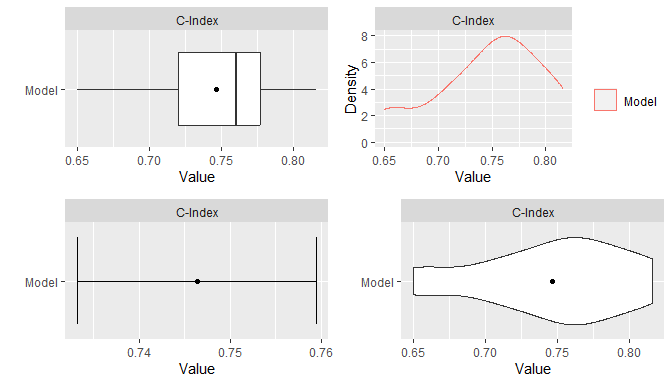## 9.6 Stratified Resampling

Stratification of cases for the construction of resampled training and test sets can be employed to help achieve balance across the sets. Stratified resampling is automatically performed if variable specification is in terms of a traditional formula or design matrix and will be done according to the response variable. For model frames and recipes, stratification variables must be defined explicitly with the strata argument to the ModelFrame() constructor or with the role_case() function. In general, strata are constructed from numeric proportions for BinomialVariate; original values for character, factor, logical, and ordered; first columns of values for matrix; original values for numeric; and numeric times within event statuses for Surv. Numeric values are stratified into quantile bins and categorical values into factor levels defined by the resampling control functions. Missing values are replaced with non-missing values sampled at random with replacement.

## Model frame with response variable stratification
mf <- ModelFrame(surv_fo, data = surv_train, strata = surv_train$y) resample(mf, model = GBMModel) ## Recipe with response variable stratification rec <- recipe(y ~ ., data = surv_train) %>% role_case(stratum = y) resample(rec, model = GBMModel) ## 9.7 Dynamic Model Parameters As discussed previously in the Model Fit and Prediction section, dynamic model parameters are evaluated at the time of model fitting and can depend on the number of observations in the fitted dataset. In the context of resampling, dynamic parameters are repeatedly evaluated at each fit of the resampled datasets. As such, their values can change based on the observations selected for training at each iteration of the resampling algorithm. ## Dynamic model parameter k = log number of training set observations resample(surv_fo, data = surv_train, model = CoxStepAICModel(k = .(log(nobs)))) ## 9.8 Model Comparisons Resampled metrics from different models can be combined for comparison with the c() function. Optional names given on the left hand side of equal operators within c() calls will be used as labels in output from the summary() and plot() functions. For comparisons of resampled output, the same control structure must be used in all associated calls to resample() to ensure that resulting model metrics are computed on the same resampled training and test sets. The combined resample output can be summarized and plotted as usual. ## Resample estimation res1 <- resample(surv_fo, data = surv_train, model = GBMModel(n.trees = 25), control = surv_means_control) res2 <- resample(surv_fo, data = surv_train, model = GBMModel(n.trees = 50), control = surv_means_control) res3 <- resample(surv_fo, data = surv_train, model = GBMModel(n.trees = 100), control = surv_means_control) ## Combine resample output for comparison (res <- c(GBM1 = res1, GBM2 = res2, GBM3 = res3)) #> --- Resample object ------------------------------------------------------------ #> #> Models: GBM1, GBM2, GBM3 #> Sampling variables: #> # A tibble: 136 × 2 #> Case Stratification$(strata)
#>    <chr> <fct>
#>  1 159   0:(2.56e+03,3.45e+03]
#>  2 179   0:(3.45e+03,5.56e+03]
#>  3 14    1:[99,1.05e+03]
#>  4 195   0:(3.45e+03,5.56e+03]
#>  5 170   0:(2.56e+03,3.45e+03]
#>  6 50    0:[1.5e+03,1.92e+03]
#>  7 118   1:(1.05e+03,3.18e+03]
#>  8 43    1:(1.05e+03,3.18e+03]
#>  9 203   0:(3.45e+03,5.56e+03]
#> 10 199   0:(3.45e+03,5.56e+03]
#> # … with 126 more rows
#>
#> === CVControl object ===
#>
#> Label: K-Fold Cross-Validation
#> Folds: 5
#> Repeats: 3

summary(res)
#> , , Metric = C-Index
#>
#>       Statistic
#> Model       Mean    Median         SD       Min       Max NA
#>   GBM1 0.7748099 0.7951389 0.04746402 0.6497797 0.8301887  0
#>   GBM2 0.7647633 0.7630332 0.05151443 0.6497797 0.8632075  0
#>   GBM3 0.7463392 0.7601810 0.05087334 0.6497797 0.8160377  0

plot(res)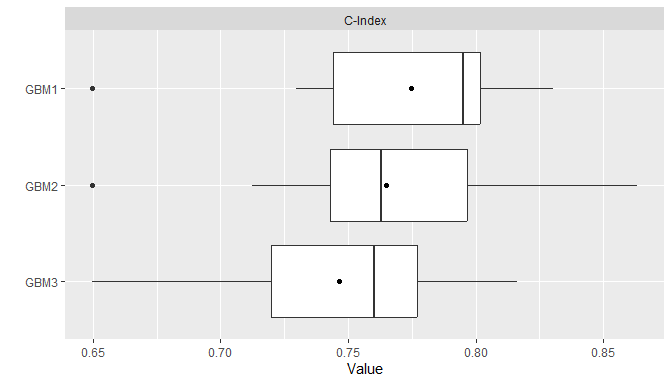Pairwise model differences for each metric can be calculated with the diff() function applied to results from a call to c(). Resulting differences can be summarized descriptively with the summary() and plot() functions and assessed for statistical significance with pairwise t-tests performed by the t.test() function. The t-test statistic for a given set of $$R$$ resampled differences is calculated as $t = \frac{\bar{x}_R}{\sqrt{F s^2_R / R}},$ where $$\bar{x}_R$$ and $$s^2_R$$ are the sample mean and variance. Statistical testing for a mean difference is then performed by comparing $$t$$ to a $$t_{R-1}$$ null distribution. The sample variance in the t statistic is known to underestimate the true variances of cross-validation mean estimators. Underestimation of these variances will lead to increased probabilities of false-positive statistical conclusions. Thus, an additional factor $$F$$ is included in the t statistic to allow for variance corrections. A correction of $$F = 1 + K / (K - 1)$$ was found by Nadeau and Bengio (2003) to be a good choice for cross-validation with $$K$$ folds and is thus used for that resampling method. The extension of this correction by Bouchaert and Frank (2004) to $$F = 1 + T K / (K - 1)$$ is used for cross-validation with $$K$$ folds repeated $$T$$ times. For other resampling methods $$F = 1$$. Below are t-test results based on the extended correction factor for 3 repeats of 5-fold cross-validation.

## Pairwise model comparisons
(res_diff <- diff(res))
#> --- PerformanceDiff object -----------------------------------------------------
#>
#> Metric: C-Index
#> Models: GBM1 - GBM2, GBM1 - GBM3, GBM2 - GBM3
#>
#> === CVControl object ===
#>
#> Label: K-Fold Cross-Validation
#> Folds: 5
#> Repeats: 3

summary(res_diff)
#> , , Metric = C-Index
#>
#>              Statistic
#> Model               Mean     Median         SD          Min        Max NA
#>   GBM1 - GBM2 0.01004663 0.01388889 0.02165165 -0.033018868 0.03973510  0
#>   GBM1 - GBM3 0.02847075 0.02903226 0.02584321 -0.009803922 0.08293839  0
#>   GBM2 - GBM3 0.01842412 0.01376147 0.01974621  0.000000000 0.05743243  0

plot(res_diff)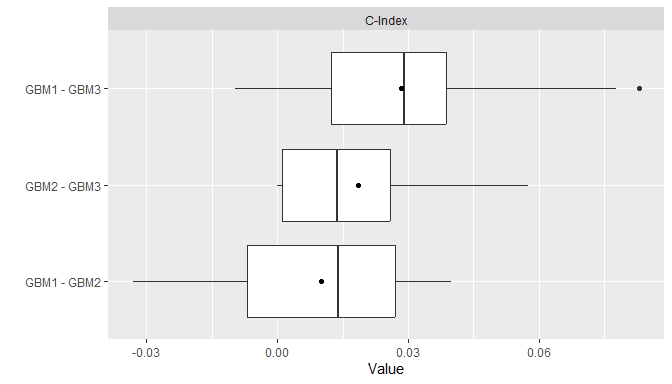t.test(res_diff)
#> --- PerformanceDiffTest object -------------------------------------------------
#>
#> Upper diagonal: mean differences (Model1 - Model2)
#> Lower diagonal: p-values
#>
#> , , Metric = C-Index
#>
#>       Model2
#> Model1      GBM1       GBM2       GBM3
#>   GBM1        NA 0.01004663 0.02847075
#>   GBM2 0.4234417         NA 0.01842412
#>   GBM3 0.2115012 0.23906539         NA

# 10 Model Predictor Effects and Diagnostics

Calculation of performance metrics on test sets or by resampling, as discussed previously, is one method of assessing model performance. Others available include measures of predictor variable importance, partial dependence plots, calibration curves comparing observed and predicted response values, and receiver operating characteristic analysis.

## 10.1 Variable Importance

The importance of predictor variables in a model fit is estimated with the varimp() function and displayed graphically with plot(). Variable importance is a relative measure of the contributions of model predictors and is scaled by default to have a maximum value of 100, where higher values represent more important variables. Model-specific implementations of variable importance are available in many cases, although their definitions may differ. In the case of a GBMModel, importance of each predictor is based on the sum of squared empirical improvements over all internal tree nodes created by splitting on that variable (Greenwell et al. 2019).

## Predictor variable importance
(vi <- varimp(surv_fit, method = "model"))
#> --- VariableImportance object --------------------------------------------------
#>             Overall
#> age       100.00000
#> thickness  78.27486
#> year       45.60724
#> ulcer      26.88075
#> sex         8.93267

plot(vi)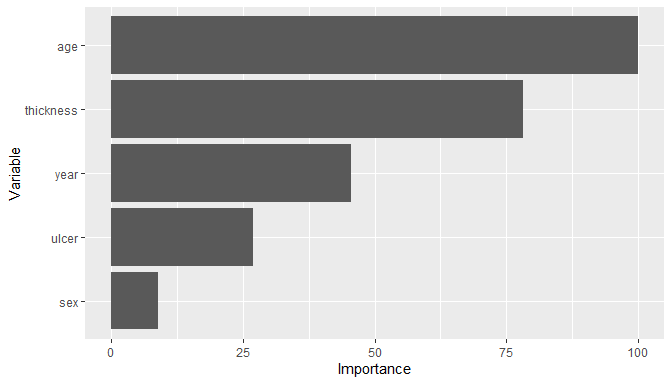In contrast, importance is based on negative log-transformed p-values for statistical models, like CoxModel, that produce them. For other models, variable importance may be defined and calculated by their underlying source packages or not defined at all, as is the case for SVMModel. Logical indicators of model-specific variable importance are given in the information displayed by model constructors and returned by modelinfo().

SVMModel
#> --- MLModelFunction object -----------------------------------------------------
#>
#> Model name: SVMModel
#> Label: Support Vector Machines
#> Package: kernlab
#> Response types: factor, numeric
#> Case weights support: FALSE
#> Missing case removal: all
#> Tuning grid: FALSE
#> Variable importance: FALSE
#>
#> Arguments:
#> function (scaled = TRUE, type = character(), kernel = c("rbfdot",
#>     "polydot", "vanilladot", "tanhdot", "laplacedot", "besseldot",
#>     "anovadot", "splinedot"), kpar = "automatic", C = 1, nu = 0.2,
#>     epsilon = 0.1, prob.model = FALSE, cache = 40, tol = 0.001,
#>     shrinking = TRUE)
#> NULL

modelinfo(SVMModel)[]$varimp #>  FALSE Variable importance can be computed with model-agnostic permutation methods (Fisher, Rudin, and Dominici 2019) as an alternative to model-specific methods. The following algorithm for permutation-based variable importance is implemented and the default method in MachineShop. • Fit a model to a training dataset. • Compute training performance. • For $$s = 1, \ldots, S$$. • Optionally sample a subset of the training set without replacement. • For predictor variable $$p = 1, \ldots, P$$. • Randomly permute the variable values. • Compute performance on the permutation set. • Compute importance as the difference or ratio between the permutation and training performances. • Reset the variable to its original values. • Return the mean or other summary statistics of importance for each variable. ## Permutation-based variable importance varimp(surv_fit) #> --- VariableImportance object -------------------------------------------------- #> Permute.mean.cindex #> age 100.000000 #> thickness 90.837696 #> ulcer 69.371728 #> year 50.130890 #> sex 6.151832 There are a number of advantages to permutation-based variable importance. In particular, it can be computed for any 1. model, 2. performance metric defined for the given response variable type, and 3. predictor variable in the original training set. Conversely, model-specific methods are not defined for some models, are generally limited to metrics implemented in their source packages, and might be computed on derived, rather than original, predictor variables. These differences can make comparisons of variable importance across classes of models difficult if not impossible. The trade-off for the advantages of a permutation-based approach is increased computation time. To speed up computations, the algorithm will run in parallel if a compatible backend is loaded as described in the Parallel Processing section. ## 10.2 Recursive Feature Elimination Recursive feature elimination (RFE) is a wrapper method of variable selection. In wrapper methods, a given model is fit to subsets of predictor variables in order to select the subset whose fit is optimal. Forward, backward, and step-wise variable selection are examples of wrapper methods. RFE is a type of backward selection in which subsets are formed from decreasing numbers of the most important predictor variables. The RFE algorithm implemented in the package-supplied rfe() function is summarized below. • Compute variable importance for all predictors. • For predictor subsets of sizes $$S = S_n > ... > S_1$$. • Eliminate predictors whose variable importance is not in the top $$S$$ by randomly permuting their values. • Compute a resampled estimate of model predictive performance. • Optionally recompute variable importance for the top $$S$$ predictors, and set importance equal to zero for those eliminated. • Select the predictor set with highest predictive performance. This RFE algorithm differs from others in that variables are “eliminated” by permuting their values rather than by removing them from the dataset. Using a permutation approach for both the elimination of variables and computation of variable importance enables application of the rfe() function to any variable specification (traditional formula, design matrix, model frame, or recipe) and any model available in the package. The syntax for rfe() is similar to resample() as illustrated in the following example. ## Recursive feature elimination (surv_rfe <- rfe(surv_fo, data = surv_train, model = GBMModel, control = surv_means_control)) #> --- TrainingStep object -------------------------------------------------------- #> #> Optimization method: Global Recursive Feature Elimination #> ModelSpecification log: #> # A tibble: 4 × 5 #> name terms selected params$size metrics$C-Index #> <chr> <list> <lgl> <int> <dbl> #> 1 ModelSpecification.1 <chr > FALSE 5 0.746 #> 2 ModelSpecification.2 <chr > FALSE 4 0.736 #> 3 ModelSpecification.3 <chr > TRUE 2 0.748 #> 4 ModelSpecification.4 <chr > FALSE 1 0.701 #> #> Selected row: 3 #> Metric: C-Index = 0.7481588 rfe_summary <- summary(surv_rfe) rfe_summary$terms[rfe_summary$selected] #> [] #>  "age" "thickness" ## 10.3 Partial Dependence Plots Partial dependence plots show the marginal effects of predictors on a response variable. Dependence for a select set of one or more predictor variables $$X_S$$ is computed as $\bar{f}_S(X_S) = \frac{1}{N}\sum_{i=1}^N f(X_S, x_{iS'}),$ where $$f$$ is a fitted prediction function and $$x_{iS'}$$ are values of the remaining predictors in a dataset of $$N$$ cases. The response scale displayed in dependence plots will depend on the response variable type: probability for predicted factors and survival probabilities, original scale for numerics, and survival time for predicted survival means. By default, dependence is computed for each selected predictor individually over a grid of 10 approximately evenly spaced values and averaged over the dataset on which the prediction function was fit. ## Partial dependence plots pd <- dependence(surv_fit, select = c(thickness, age)) plot(pd)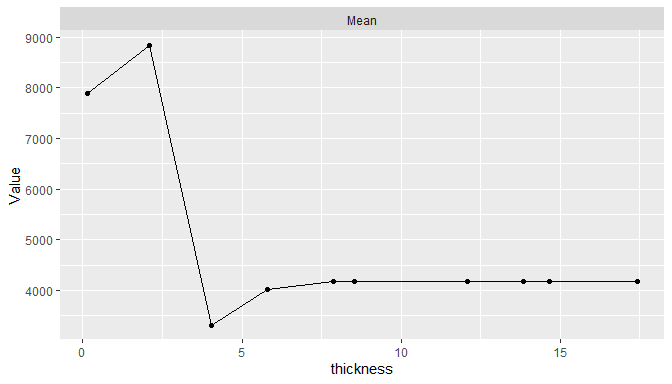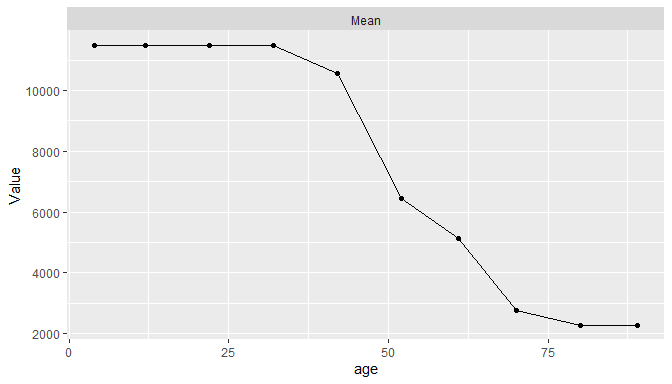Estimated predictor effects are marginal in that they are averaged over the remaining variables, whose distribution depends on the population represented by the dataset. Consequently, partial dependence plots for a given model can vary across datasets and populations. The package allows averaging over different datasets to estimate marginal effects in other case populations, over different numbers of predictor values, and over quantile spacing of the values. pd <- dependence(surv_fit, data = surv_test, select = thickness, n = 20, intervals = "quantile") plot(pd)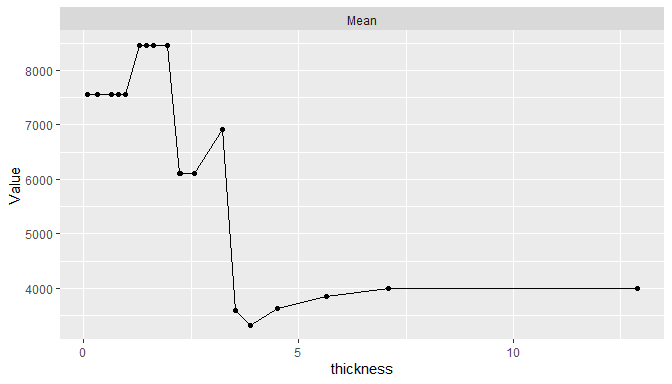In addition, dependence may be computed for combinations of multiple predictors to examine interaction effects and for summary statistics other than the mean. ## 10.4 Calibration Curves Agreement between model-predicted and observed values can be visualized with calibration curves. Calibration curves supplement individual performance metrics with information on model fit in different regions of predicted values. They also provide more direct assessment of agreement than some performance metrics, like ROC AUC, that do not account for scale and location differences. In the construction of binned calibration curves, cases are partitioned into equal-width intervals according to their (resampled) predicted responses. Mean observed responses are then calculated within each of the bins and plotted on the vertical axis against the bin midpoints on the horizontal axis. ## Binned calibration curves cal <- calibration(res_probs, breaks = 10) plot(cal, se = TRUE)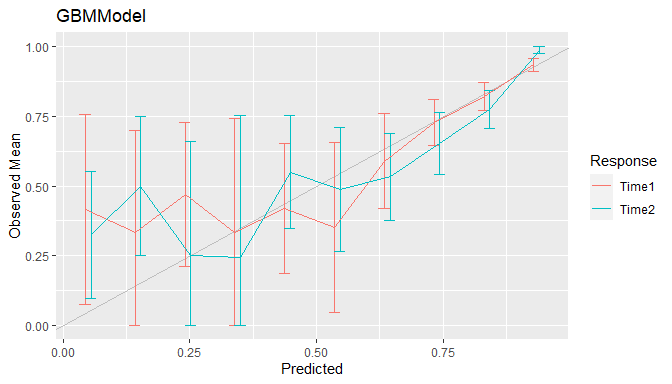As an alternative to discrete bins, curves can be smoothed by setting breaks = NULL to compute weighted averages of observed values. Smoothing has the advantage of producing more precise curves by including more observed values in the calculation at each predicted value. ## Smoothed calibration curves cal <- calibration(res_probs, breaks = NULL) plot(cal)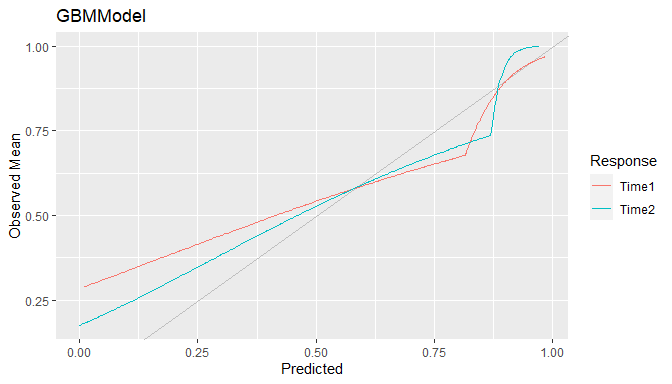Calibration curves close to the 45$$^\circ$$ line represent agreement between observed and predicted responses and a model that is said to be well calibrated. ## 10.5 Confusion Matrices Confusion matrices of cross-classified observed and predicted categorical responses are available with the confusion() function. They can be constructed with predicted class membership or with predicted class probabilities. In the latter case, predicted class membership is derived from predicted probabilities according to a probability cutoff value for binary factors (default: cutoff = 0.5) and according to the class with highest probability for factors with more than two levels. ## Confusion matrices (conf <- confusion(res_probs, cutoff = 0.7)) #> --- ConfusionList object ------------------------------------------------------- #> #> ===$GBMModel.Time1 ============================================================
#> === BinaryConfusionMatrix object ===
#>          Observed
#> Predicted         0         1
#>         0 236.02496  38.97504
#>         1  59.34328  73.65672
#>
#> === $GBMModel.Time2 ============================================================ #> === BinaryConfusionMatrix object === #> Observed #> Predicted 0 1 #> 0 170.20031 34.79969 #> 1 82.12352 120.87648 plot(conf)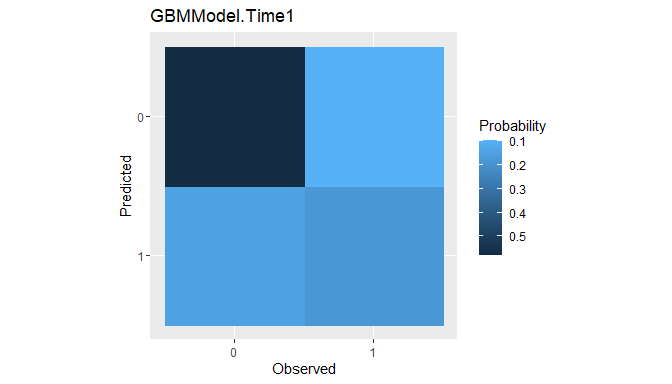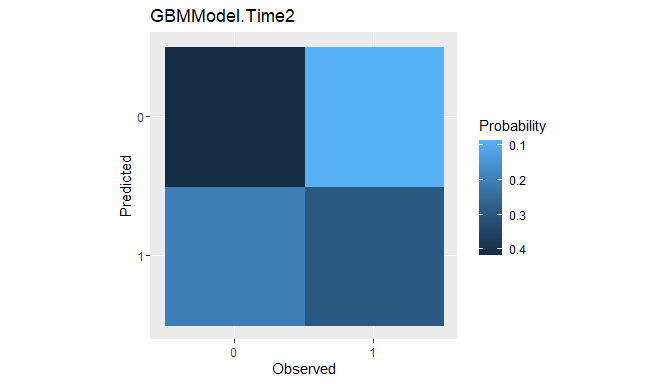Confusion matrices are the data structure upon which many of the performance metrics described earlier for factor predictor variables are based. Metrics commonly reported for confusion matrices are generated by the summary() function. ## Summary performance metrics summary(conf) #> ---$GBMModel.Time1 ------------------------------------------------------------
#> Number of responses: 408
#> Accuracy (SE): 0.7590237 (0.02117311)
#> Majority class: 0.7239418
#> Kappa: 0.4290466
#>
#>                     0         1
#> Observed    0.7239418 0.2760582
#> Predicted   0.6740196 0.3259804
#> Agreement   0.5784925 0.1805312
#> Sensitivity 0.7990871 0.6539605
#> Specificity 0.6539605 0.7990871
#> PPV         0.8582726 0.5538099
#> NPV         0.5538099 0.8582726
#>
#> --- $GBMModel.Time2 ------------------------------------------------------------ #> Number of responses: 408 #> Accuracy (SE): 0.7134235 (0.02238535) #> Majority class: 0.6184408 #> Kappa: 0.4261807 #> #> 0 1 #> Observed 0.6184408 0.3815592 #> Predicted 0.5024510 0.4975490 #> Agreement 0.4171576 0.2962659 #> Sensitivity 0.6745312 0.7764610 #> Specificity 0.7764610 0.6745312 #> PPV 0.8302454 0.5954506 #> NPV 0.5954506 0.8302454 Summaries can also be obtained with the performance() function for default or use-specified metrics. ## Confusion matrix-specific metrics metricinfo(conf) %>% names #>  "accuracy" "f_score" "fnr" "fpr" "kappa2" #>  "npv" "ppr" "ppv" "precision" "recall" #>  "roc_index" "sensitivity" "specificity" "tnr" "tpr" ## User-specified metrics performance(conf, metrics = c("Accuracy" = accuracy, "Sensitivity" = sensitivity, "Specificity" = specificity)) #> ---$GBMModel.Time1 ------------------------------------------------------------
#>    Accuracy Sensitivity Specificity
#>   0.7590237   0.6539605   0.7990871
#>
#> --- GBMModel.Time2 ------------------------------------------------------------ #> Accuracy Sensitivity Specificity #> 0.7134235 0.7764610 0.6745312 ## 10.6 Performance Curves Tradeoffs between correct and incorrect classifications of binary responses, across the range of possible cutoff probabilities, can be studied with performance curves. In general, any two binary response metrics may be specified for the construction of a performance curve. ### 10.6.1 ROC Curves Receiver operating characteristic (ROC) curves are one example in which true positive rates (sensitivity) are plotted against false positive rates (1 - specificity) (Fawcett 2006). True positive rate (TPR) and false positive rate (FPR) are defined as \begin{aligned} TPR &= \text{sensitivity} = \Pr(\hat{p} > c \mid D^+) \\ FPR &= 1 - \text{specificity} = \Pr(\hat{p} > c \mid D^-), \end{aligned} where $$\hat{p}$$ is the model-predicted probability of being positive, $$0 \le c \le 1$$ is a probability cutoff value for classification as positive or negative, and $$D^+/D^-$$ is positive/negative case status. ROC curves show tradeoffs between the two rates over the range of possible cutoff values. Higher curves are indicative of better predictive performance. ## ROC curves roc <- performance_curve(res_probs) plot(roc, diagonal = TRUE)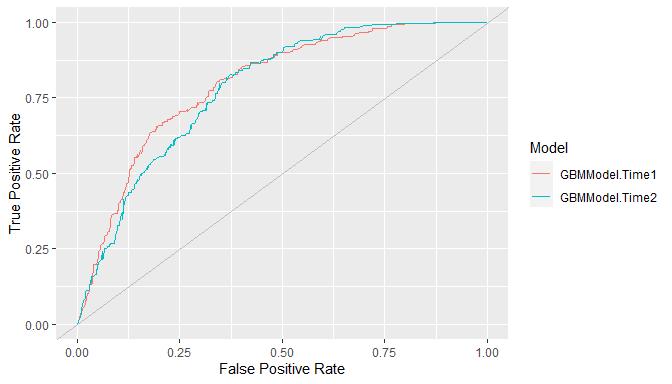ROC curves show the relation between the two rates being plotted but not their relationships with specific cutoff values. The latter may be helpful for the selection of a cutoff to apply in practice. Accordingly, separate plots of each rate versus the range of possible cutoffs are available with the type = "cutoffs" option. plot(roc, type = "cutoffs")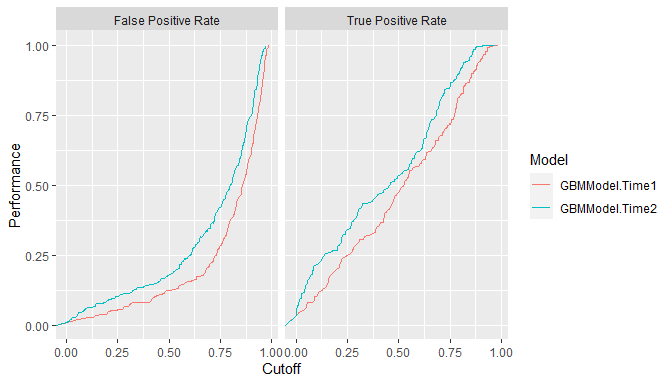Area under the ROC curve (ROC AUC) is an overall measure of model predictive performance. It is interpreted as the probability that a randomly selected positive case will have a higher predicted value than a randomly selected negative case. AUC values of 0.5 and 1.0 indicate chance and perfect concordance between predicted probabilities and observed responses. auc(roc) #> Model: GBMModel.Time1 #>  0.7966402 #> ------------------------------------------------------------ #> Model: GBMModel.Time2 #>  0.7818386 ### 10.6.2 Precision Recall Curves Precision recall curves plot precision (positive predictive value) against recall (sensitivity) (Davis and Goadrich 2006), where \begin{aligned} \text{precision} &= PPV = \Pr(D^+ \mid \hat{p} > c) \\ \text{recall} &= \text{sensitivity} = \Pr(\hat{p} > c \mid D^+). \end{aligned} These curves tend to be used when primary interest lies in detecting positive cases and such cases are rare. ## Precision recall curves pr <- performance_curve(res_probs, metrics = c(precision, recall)) plot(pr)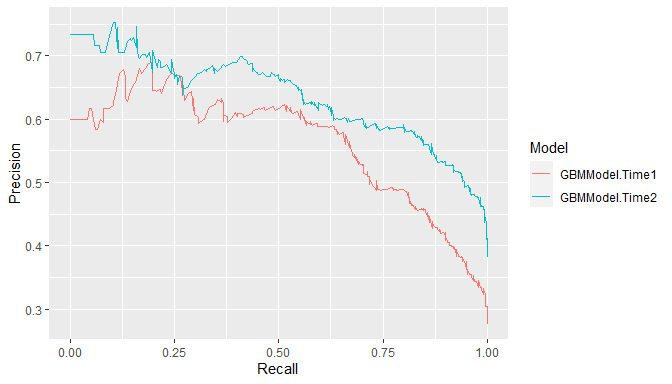auc(pr) #> Model: GBMModel.Time1 #>  0.562425 #> ------------------------------------------------------------ #> Model: GBMModel.Time2 #>  0.6359023 ### 10.6.3 Lift Curves Lift curves depict the rate at which positive cases are found as a function of the proportion predicted to be positive in the population. In particular, they plot true positive rate (sensitivity) against positive prediction rate (PPR) for all possible classification probability cutoffs, where \begin{aligned} TPR &= \Pr(\hat{p} > c \mid D^+) \\ PPR &= \Pr(\hat{p} > c). \end{aligned} Models more efficient (lower cost) at identifying positive cases find them at a higher proportion ($$TPR$$) while predicting fewer in the overall population to be positive ($$PPR$$). In other words, higher lift curves are signs of model efficiency. ## Lift curves lf <- lift(res_probs) plot(lf, find = 0.75)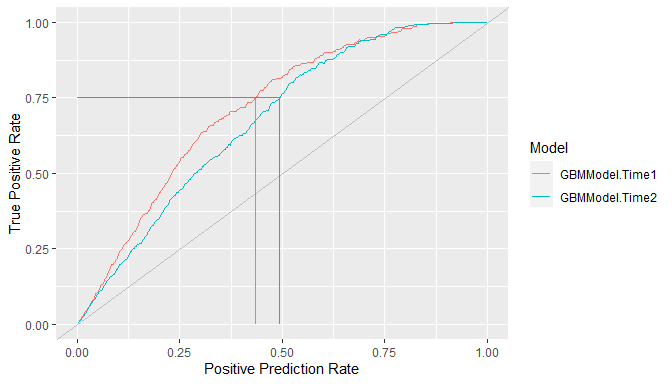# 11 Modeling Strategies Model development often involves the comparison of multiple models from a candidate set for the purpose of selecting a final one. Models in the set may differ with respect to their predictor variables, preprocessing steps and parameters, and model types and parameters. Complex model selection strategies for sets that involve one or more of these differences can be implemented with the MachineShop package. Implementation is achieved with a straightforward syntax based on the meta-input and meta-model functions listed in the table below and with resampling, including nested resampling, conducted automatically for model selection and predictive performance evaluation. Parameter Grid Tuning Candidate Set Selection Ensemble Learning TunedInput() SelectedInput() StackedModel() TunedModel() SelectedModel() SuperModel() These meta-functions fall into three main categories: 1) tuning of a given input or model over a grid of parameter values, 2) selection from an arbitrary set of different inputs or models, or 3) combining multiple models into an ensemble learner. In the context of these strategies, an input may be a formula, design matrix, model frame, or preprocessing recipe. The meta-input and meta-model functions themselves return input and model class objects, respectively. Combinations and multiple levels of nesting of meta-functions, inputs, and models are allowed. For example, StackedModel() and SuperModel() may consist of TunedModel and other model objects. SelectedModel() can select among mixes of TunedModel, ensemble model, and other model objects. Likewise, TunedInput objects, along with other inputs, may be nested within SelectedInput(). Furthermore, selection and tuning of both inputs and models can be performed simultaneously. These and other possibilities are illustrated in the following sections. ## 11.1 Inputs Inputs to model fitting functions define the predictor and response variables and the dataset containing their values. These can be specified with traditional formula and dataset pairs, design matrix and response variable pairs, model frames, and preprocessing recipes. The package supports (1) tuning of an input over a grid of parameter values and (2) selection of inputs from candidate sets that differ with respect to their predictors or their preprocessing steps and parameters. ### 11.1.1 Input Tuning Preprocessing recipes may have step with parameters that affect predictive performance. Steps can be tuned over a grid of parameter values with TunedInput() to select the best performing values. Calls to TunedInput() return an input object that may be trained on data with the fit() function or evaluated for predictive performance with resample(). As an example, a principal components analysis (PCA) step could be included in a preprocessing recipe for tuning over the number of components to retain in the final model. Such a recipe is shown below accompanied by a call to expand_steps() to construct a tuning grid. The grid parameter num_comp and name PCA correspond to the argument and id of the step_pca() function to which the values 1:3 apply. The recipe and grid may then be passed to TunedInput() for model fitting. ## Preprocessing recipe with PCA steps pca_rec <- recipe(y ~ ., data = surv_train) %>% role_case(stratum = y) %>% step_center(all_predictors()) %>% step_scale(all_predictors()) %>% step_pca(all_predictors(), id = "PCA") ## Tuning grid of number of PCA components pca_grid <- expand_steps( PCA = list(num_comp = 1:3) ) ## Tuning specification tun_rec <- TunedInput(pca_rec, grid = pca_grid) From the fit, the resulting model can be extracted with as.MLModel(). The output shows that one principal component was selected. Resample estimation of predictive performance is applied to a TunedInput specification for the selection. The default resampling method is cross-validation. Other methods, performance metrics, and selection statistics can be supplied to the TunedInput() arguments. ## Input-tuned model fit and final trained model model_fit <- fit(tun_rec, model = GBMModel) as.MLModel(model_fit) #> --- MLModel object ------------------------------------------------------------- #> #> Model name: GBMModel #> Label: Trained Generalized Boosted Regression #> Package: gbm #> Response types: factor, numeric, PoissonVariate, Surv #> Case weights support: TRUE #> Missing case removal: response #> Tuning grid: TRUE #> Variable importance: TRUE #> #> Parameters: #> List of 5 #> n.trees          : num 100
#>  $interaction.depth: num 1 #>$ n.minobsinnode   : num 10
#>  $shrinkage : num 0.1 #>$ bag.fraction     : num 0.5
#>
#> === $TrainingStep1 ============================================================= #> === TrainingStep object === #> #> Optimization method: Grid Search #> TunedModelRecipe log: #> # A tibble: 3 × 4 #> name selected params$PCA$num_comp metrics$C-Index
#>   <chr>         <lgl>                  <int>             <dbl>
#> 1 ModelRecipe.1 TRUE                       1             0.740
#> 2 ModelRecipe.2 FALSE                      2             0.720
#> 3 ModelRecipe.3 FALSE                      3             0.725
#>
#> Selected row: 1
#> Metric: C-Index = 0.7399641

### 11.1.2 Input Selection

Selection of recipes with different steps or predictors can be conducted with SelectedInput().

## Preprocessing recipe without PCA steps
rec1 <- recipe(y ~ sex + age + year + thickness + ulcer, data = surv_train) %>%
role_case(stratum = y)
rec2 <- recipe(y ~ sex + age + year, data = surv_train) %>%
role_case(stratum = y)

## Selection among recipes with and without PCA steps
sel_rec <- SelectedInput(
rec1,
rec2,
TunedInput(pca_rec, grid = pca_grid)
)

In this case, the third recipe with PCA steps is selected.

## Input-selected model fit and model
model_fit <- fit(sel_rec, model = GBMModel)
as.MLModel(model_fit)
#> --- MLModel object -------------------------------------------------------------
#>
#> Model name: GBMModel
#> Label: Trained Generalized Boosted Regression
#> Package: gbm
#> Response types: factor, numeric, PoissonVariate, Surv
#> Case weights support: TRUE
#> Missing case removal: response
#> Tuning grid: TRUE
#> Variable importance: TRUE
#>
#> Parameters:
#> List of 5
#>  $n.trees : num 100 #>$ interaction.depth: num 1
#>  $n.minobsinnode : num 10 #>$ shrinkage        : num 0.1
#>  $bag.fraction : num 0.5 #> #> ===$TrainingStep1 =============================================================
#> === TrainingStep object ===
#>
#> Optimization method: Grid Search
#> SelectedModelRecipe log:
#> # A tibble: 3 × 4
#>   name             selected params$id metrics$C-Index
#>   <chr>            <lgl>    <chr>                  <dbl>
#> 1 ModelRecipe.1    FALSE    input.azpi             0.761
#> 2 ModelRecipe.2    FALSE    input.aLHo             0.643
#> 3 TunedModelRecipe TRUE     input.W4KN             0.796
#>
#> Selected row: 3
#> Metric: C-Index = 0.7960841
#>
#> === $TrainingStep2 ============================================================= #> === TrainingStep object === #> #> Optimization method: Grid Search #> TunedModelRecipe log: #> # A tibble: 3 × 4 #> name selected params$PCA$num_comp metrics$C-Index
#>   <chr>         <lgl>                  <int>             <dbl>
#> 1 ModelRecipe.1 TRUE                       1             0.740
#> 2 ModelRecipe.2 FALSE                      2             0.720
#> 3 ModelRecipe.3 FALSE                      3             0.725
#>
#> Selected row: 1
#> Metric: C-Index = 0.7399641

Selection can also be performed among traditional formulas, design matrices, or model frames.

## Traditional formulas
fo1 <- y ~ sex + age + year + thickness + ulcer
fo2 <- y ~ sex + age + year

## Selection among formulas
sel_fo <- SelectedInput(fo1, fo2, data = surv_train)

## Input-selected model fit and final trained model
model_fit <- fit(sel_fo, model = GBMModel)
as.MLModel(model_fit)

In the previous examples, selection of different inputs was performed with the same model (GBMModel). Selection among different combinations of inputs and models is supported with the ModelSpecification() constructor.

## Different combinations of inputs and models
sel_mfo <- SelectedInput(
ModelSpecification(fo1, data = surv_train, model = CoxModel),
ModelSpecification(fo2, data = surv_train, model = GBMModel)
)

## Input-selected model fit and final trained model
model_fit <- fit(sel_mfo)
as.MLModel(model_fit)

## 11.2 Models

Models define the functional relationships between predictor and response variables from a given set of inputs.

### 11.2.1 Model Tuning

Many of the package-supplied modeling functions have arguments, or tuning parameters, that control aspects of their model fitting algorithms. For example, GBMModel parameters n.trees and interaction.depth control the number of decision trees to fit and the maximum tree depths. When called with a TunedModel, the fit() function performs model fitting over a grid of parameter values and returns the model with the optimal values. Optimality is determined based on the first performance metric of the metrics argument to TunedModel() if given or the first default metric of the performance() function otherwise. Argument grid additionally controls the construction of grid values and can be a single integer or vector of integers whose positions or names match the parameters in a model’s pre-defined tuning grid if one exists and which specify the number of values used to construct the grid. Pre-defined TunedModel grids can be extract and viewed apart from model fitting with expand_modelgrid(). As shown in the output below, as.MLModel() will extract a tuned model from fit results for viewing of the tuning parameter grid values, the names of models fit to each, all calculated metrics, the final model selected, the metric upon which its selection was based, and its parameters.

## Tune over automatic grid of model parameters
model_fit <- fit(surv_fo, data = surv_train,
model = TunedModel(
GBMModel,
grid = 3,
control = surv_means_control,
metrics = c("CIndex" = cindex, "RMSE" = rmse)
))
(trained_model <- as.MLModel(model_fit))
#> --- MLModel object -------------------------------------------------------------
#>
#> Model name: GBMModel
#> Label: Trained Generalized Boosted Regression
#> Package: gbm
#> Response types: factor, numeric, PoissonVariate, Surv
#> Case weights support: TRUE
#> Missing case removal: response
#> Tuning grid: TRUE
#> Variable importance: TRUE
#>
#> Parameters:
#> List of 5
#>  $n.trees : int 50 #>$ interaction.depth: int 1
#>  $n.minobsinnode : num 10 #>$ shrinkage        : num 0.1
#>  $bag.fraction : num 0.5 #> #> ===$TrainingStep1 =============================================================
#> === TrainingStep object ===
#>
#> Optimization method: Grid Search
#> TunedModel log:
#> # A tibble: 9 × 4
#>   name       selected params$n.trees$interaction.depth metrics$CIndex$RMSE
#>   <chr>      <lgl>             <int>              <int>          <dbl>  <dbl>
#> 1 GBMModel.1 TRUE                 50                  1          0.765  3869.
#> 2 GBMModel.2 FALSE                50                  2          0.750  5450.
#> 3 GBMModel.3 FALSE                50                  3          0.739  7877.
#> 4 GBMModel.4 FALSE               100                  1          0.746  3919.
#> 5 GBMModel.5 FALSE               100                  2          0.730  5262.
#> 6 GBMModel.6 FALSE               100                  3          0.720 10596.
#> 7 GBMModel.7 FALSE               150                  1          0.726  4167.
#> 8 GBMModel.8 FALSE               150                  2          0.712  5428.
#> 9 GBMModel.9 FALSE               150                  3          0.699 16942.
#>
#> Selected row: 1
#> Metric: CIndex = 0.7647633

Grid values may also be given as a TuningGrid function, function name, or object; ParameterGrid object; or data frame containing parameter values at which to evaluate the model, such as that returned by expand_params().

## Tune over randomly sampled grid points
fit(surv_fo, data = surv_train,
model = TunedModel(
GBMModel,
grid = TuningGrid(size = 100, random = 10),
control = surv_means_control
))

## Tune over user-specified grid points
fit(surv_fo, data = surv_train,
model = TunedModel(
GBMModel,
grid = expand_params(n.trees = c(25, 50, 100),
interaction.depth = 1:3),
control = surv_means_control
))

Statistics summarizing the resampled performance metrics across all tuning parameter combinations can be obtained with the summary() and performance()functions.

summary(trained_model)
#> --- $TrainingStep1 ------------------------------------------------------------- #> # A tibble: 9 × 4 #> name selected params$n.trees $interaction.depth metrics$CIndex  $RMSE #> <chr> <lgl> <int> <int> <dbl> <dbl> #> 1 GBMModel.1 TRUE 50 1 0.765 3869. #> 2 GBMModel.2 FALSE 50 2 0.750 5450. #> 3 GBMModel.3 FALSE 50 3 0.739 7877. #> 4 GBMModel.4 FALSE 100 1 0.746 3919. #> 5 GBMModel.5 FALSE 100 2 0.730 5262. #> 6 GBMModel.6 FALSE 100 3 0.720 10596. #> 7 GBMModel.7 FALSE 150 1 0.726 4167. #> 8 GBMModel.8 FALSE 150 2 0.712 5428. #> 9 GBMModel.9 FALSE 150 3 0.699 16942. performance(trained_model) %>% lapply(summary) #>$TrainingStep1
#> , , Metric = CIndex
#>
#>             Statistic
#> Model             Mean    Median         SD       Min       Max NA
#>   GBMModel.1 0.7647633 0.7630332 0.05151443 0.6497797 0.8632075  0
#>   GBMModel.2 0.7502765 0.7549020 0.04636802 0.6688742 0.8349057  0
#>   GBMModel.3 0.7388766 0.7500000 0.04594197 0.6621622 0.8197425  0
#>   GBMModel.4 0.7463392 0.7601810 0.05087334 0.6497797 0.8160377  0
#>   GBMModel.5 0.7301734 0.7345133 0.04361416 0.6621622 0.7847222  0
#>   GBMModel.6 0.7199483 0.7122642 0.05168649 0.6216216 0.7889908  0
#>   GBMModel.7 0.7263693 0.7385321 0.05069831 0.6351351 0.7896996  0
#>   GBMModel.8 0.7121314 0.7169811 0.05331152 0.6199095 0.7847222  0
#>   GBMModel.9 0.6994678 0.7156863 0.05314909 0.6081081 0.7639485  0
#>
#> , , Metric = RMSE
#>
#>             Statistic
#> Model             Mean    Median        SD      Min       Max NA
#>   GBMModel.1  3868.629  3321.249  1431.839 2123.875  7401.789  0
#>   GBMModel.2  5450.093  4741.129  2438.173 2385.426 11695.273  0
#>   GBMModel.3  7877.487  5827.739  5036.837 4192.463 20009.854  0
#>   GBMModel.4  3919.126  3535.514  1454.999 2373.413  7558.280  0
#>   GBMModel.5  5262.122  5052.969  1900.353 2280.193  8212.727  0
#>   GBMModel.6 10595.888  8308.733  7057.327 4996.420 28610.367  0
#>   GBMModel.7  4167.128  3789.596  1115.371 2587.440  6701.087  0
#>   GBMModel.8  5427.518  6129.309  2313.742 2039.894  9212.369  0
#>   GBMModel.9 16941.696 10865.160 16841.774 4914.743 71150.177  0

Line plots of tuning results display the resampled metric means, or another statistic specified with the stat argument, versus the first tuning parameter values and with lines grouped according to the remaining parameters, if any.

plot(trained_model, type = "line")
#> $TrainStep1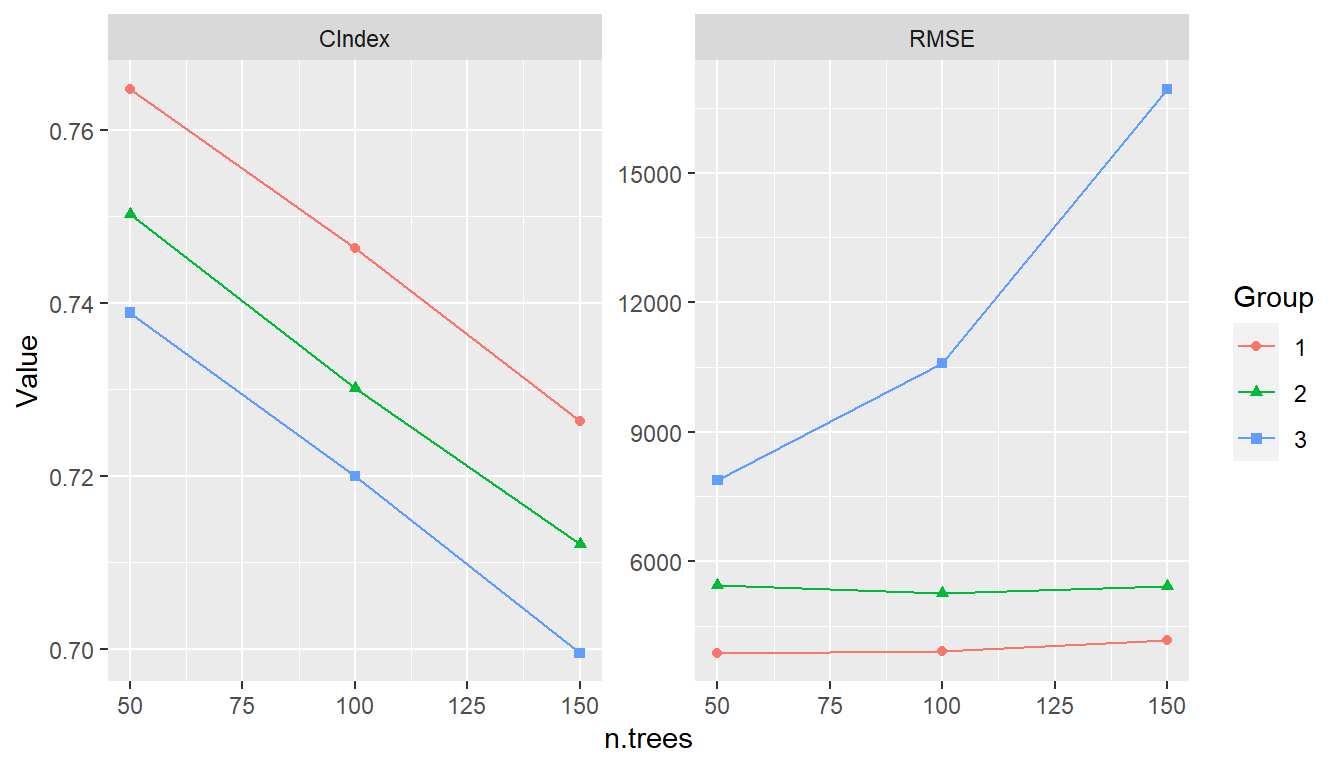### 11.2.2 Model Selection Model selection can be conducted by calling fit() with a SelectedModel to automatically choose from any combination of models and model parameters. Selection has as a special case the just-discussed tuning of a single model over a grid of parameter values. Combinations of model functions, function names, or objects can be supplied to SelectedModel() in order to define sets of candidate models from which to select. An expand_model() helper function is additionally available to expand a model over a grid of tuning parameters for inclusion in the candidate set if so desired. ## Model interface for model selection sel_model <- SelectedModel( expand_model(GBMModel, n.trees = c(50, 100), interaction.depth = 1:2), GLMNetModel(lambda = 0.01), CoxModel, SurvRegModel ) ## Fit the selected model fit(surv_fo, data = surv_train, model = sel_model) Selection may also be performed over candidate sets that include tuned models. For instance, the SelectedModel() function is applicable to sets containing different classes of models each individually tuned over a grid of parameters. ## Model interface for selection among tuned models sel_tun_model <- SelectedModel( TunedModel(GBMModel, control = surv_means_control), TunedModel(GLMNetModel, control = surv_means_control), TunedModel(CoxModel, control = surv_means_control) ) ## Fit the selected tuned model fit(surv_fo, data = surv_train, model = sel_tun_model) ## 11.3 Ensemble Learning Ensemble learning models combine $$m = 1, \ldots, M$$ base models as a strategy to improve predictive performance. Two methods implemented in MachineShop are stacked regression (Breiman 1996) and super learners (van der Laan, Polley, and Hubbard 2007). Stacked regression fits a linear combination of predictions from specified base learners to produce a prediction function of the form $\hat{f}(x) = \sum_{m=1}^M \hat{w}_m \hat{f}_m(x).$ Stacking weights $$w$$ are estimated by (constrained) least squares regression of case responses $$y_i$$ on predictions $$\hat{f}_m^{-\kappa(i)}(x_i)$$ from learners fit to data subsamples $$-\kappa(i)$$ not containing the corresponding cases. In particular, they are obtained as the solution $\hat{w} = \underset{w}{\operatorname{argmin}} \sum_{i=1}^{N}\left(y_i - \sum_{m=1}^{M} w_m \hat{f}_m^{-\kappa(i)}(x_i) \right)^2$ subject to the constraints that all $$w_m \ge 0$$ and $$\sum_m w_m = 1$$. K-fold cross-validation is the default subsampling method employed in the estimation, with the other resampling methods provided by the package available as options. Survival outcomes are handled with a modified version of the stacked regression algorithm in which • minimization of least squares is replaced by maximization of Harrell’s concordance index (1982) to accommodate censoring, and • prediction can only be performed on the same response type used for the model fit; i.e., either survival means or survival probabilities at given follow-up times. Super learners are a generalization of stacked regression that fit a specified model, such as GBMModel, to case responses $$y_i$$, base learner predictions $$\hat{f}_m^{-\kappa(i)}(x_i)$$, and optionally also to the original predictor variables $$x_i$$. Given below are examples of a stacked regression and super learner each fit with gradient boosted, random forest, and Cox regression base learners. A separate gradient boosted model is used as the super learner in the latter. ## Stacked regression stackedmodel <- StackedModel(CoxModel, CForestModel, GLMBoostModel) res_stacked <- resample(surv_fo, data = surv_train, model = stackedmodel) summary(res_stacked) #> Statistic #> Metric Mean Median SD Min Max NA #> C-Index 0.7294869 0.762963 0.1275484 0.5194805 0.8556701 0 ## Super learner supermodel <- SuperModel(CoxModel, CForestModel, GLMBoostModel, model = GBMModel) res_super <- resample(surv_fo, data = surv_train, model = supermodel) summary(res_super) #> Statistic #> Metric Mean Median SD Min Max NA #> C-Index 0.7534803 0.8325472 0.1243104 0.5748899 0.8454545 0 ## 11.4 Methodology Combinations and multiple levels of nested meta-functions, inputs, and models are possible. If model fitting involves a single meta-function, performances of the inputs or models under consideration are estimated with standard resampling, and the best performing model is returned. Nestings of meta-functions are trained with nested resampling. Consider the example below in which training involves input tuning and model selection. In particular, a preprocessing recipe is tuned over the number of predictor-derived principal components and model selection is of an untuned GBMModel, a tuned GBMModel, and a StackedModel. ## Preprocessing recipe with PCA steps pca_rec <- recipe(y ~ ., data = surv_train) %>% role_case(stratum = y) %>% step_center(all_predictors()) %>% step_scale(all_predictors()) %>% step_pca(all_predictors(), id = "PCA") ## Tuning grid of number of PCA components pca_grid <- expand_steps( PCA = list(num_comp = 1:3) ) ## Input specification tun_rec <- TunedInput(pca_rec, grid = pca_grid) ## Model specification sel_model <- SelectedModel( GBMModel, TunedModel(GBMModel), StackedModel(CoxModel, TunedModel(CForestModel), TunedModel(GBMModel)) ) ## Model fit and final trained model model_fit <- fit(tun_rec, model = sel_model) as.MLModel(model_fit) Model fitting proceeds with instances of the specified model selection nested within each of the input tuning grid parameter values. Tuning of GBMModel and construction of StackedModel are further nested within the model selection, with tuning of CForestModel and GBMModel nested within StackedModel. Altogether, there are four levels of meta-input and meta-model functions in the hierarchy.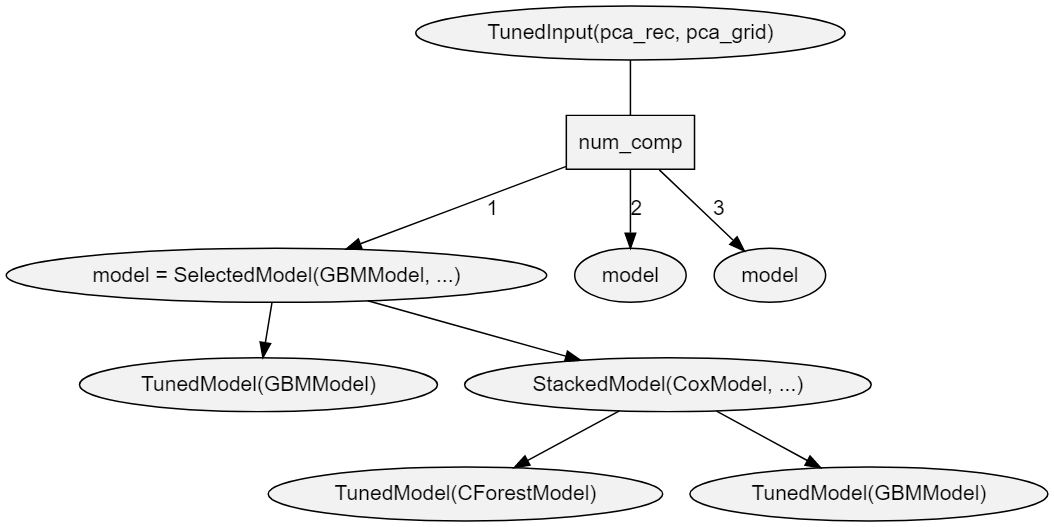Each meta-function is fit based on resample estimation (default: cross-validation) of predictive performance. When one meta-function is nested within another, nested resampling is employed, as illustrated in the figure below.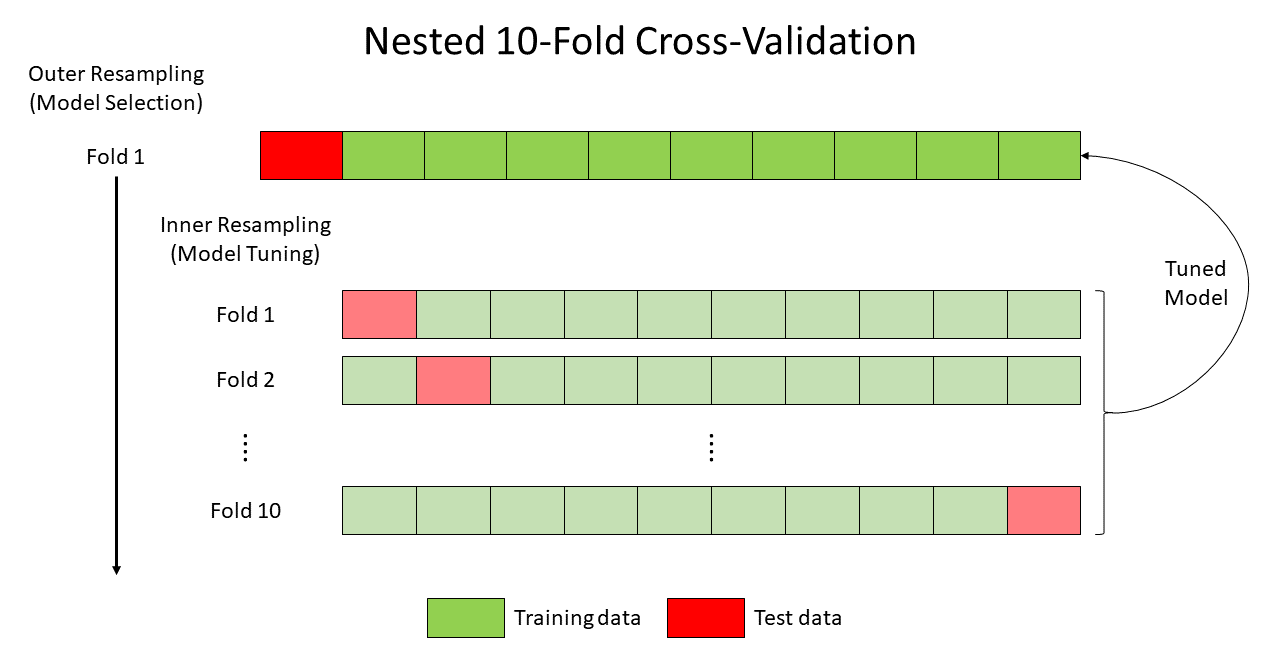Nesting of resampling routines is repeated recursively when a fit involves multiple levels of nested meta-functions. For example, predictive performance estimation for the training of TunedInput(pca_rec, grid = pca_grid) involves up to three nested meta functions: SelectedModel(…) → StackedModel(…) → TunedModel(CForestModel). For this relationship, an outer and three nested inner resampling loops are executed as follows. First, CForestModel is tuned at the third inner resampling loop. Second, the tuned model is passed to the second inner loop for construction of StackedModel. Third, the constructed model is passed to the first inner loop for model selection from the candidate set. Finally, the selected model is passed to the outer loop for tuning of the preprocessing recipe. Based on resample performance estimation of the entire input/model specification, one principal component is selected for the GBMModel. #> ===$TrainingStep1 =============================================================
#> === TrainingStep object ===
#>
#> Optimization method: Grid Search
#> TunedModelRecipe log:
#> # A tibble: 3 × 4
#>   name          selected params$PCA$num_comp metrics$C-Index #> <chr> <lgl> <int> <dbl> #> 1 ModelRecipe.1 TRUE 1 0.741 #> 2 ModelRecipe.2 FALSE 2 0.730 #> 3 ModelRecipe.3 FALSE 3 0.702 #> #> Selected row: 1 #> Metric: C-Index = 0.7405534 In order to identify and return a final model fitted to the entire input data, the hierarchy is traversed from top to bottom along the path determined by the choice at each node. Steps along the path are labeled TrainStep1 and TrainStep2 in the output. As seen above in TrainStep1, one principal component is first selected for the tuned input. Using an input recipe with one principal component, the entire dataset is refit at TrainStep2 to finally select GBMModel. #> ===$TrainingStep2 =============================================================
#> === TrainingStep object ===
#>
#> Optimization method: Grid Search
#> SelectedModel log:
#> # A tibble: 3 × 4
#>   name         selected params$id metrics$C-Index
#>   <chr>        <lgl>    <chr>                  <dbl>
#> 1 GBMModel     TRUE     model.1mQk             0.740
#> 2 TunedModel   FALSE    model.hRF5             0.735
#> 3 StackedModel FALSE    model.CYj0             0.653
#>
#> Selected row: 1
#> Metric: C-Index = 0.7399641

After the series of training steps reaches the bottom of its hierarchy, the final model is fitted to the entire dataset and returned.

#> --- MLModel object -------------------------------------------------------------
#>
#> Model name: GBMModel
#> Label: Trained Generalized Boosted Regression
#> Package: gbm
#> Response types: factor, numeric, PoissonVariate, Surv
#> Case weights support: TRUE
#> Missing case removal: response
#> Tuning grid: TRUE
#> Variable importance: TRUE
#>
#> Parameters:
#> List of 5
#>  $n.trees : num 100 #>$ interaction.depth: num 1
#>  $n.minobsinnode : num 10 #>$ shrinkage        : num 0.1
#>  $bag.fraction : num 0.5 Generalization performance of the entire process can be estimated with a call to resample(). ## Generalization performance of the modeling strategy resample(tun_rec, model = sel_model) There is no conceptual limit to the number of nested inputs and models that can be specified with the package. However, there are some practical issues to consider. Computational Expense Computational expense of nested resampling increases exponentially. For instance, execution of r levels of a nested 10-fold cross-validation algorithm is an O(10r) operation. Runtimes can be decreased by registering multiple cores to run the resampling algorithms in parallel. However, the exponential increase in computational complexity quickly outpaces the number of available cores. Data Reduction Training data is reduced at each subsequent resampling level. For 10-fold cross-validation and a training set of N total cases, there will be 0.9r cases available at each fold of the rth resampling algorithm. Bootstrapping could be used, as an alternative to cross-validation, to ensure N cases at each resampling level. However, the number of unique cases at level r will be decreased to approximately N(2/3)r. # 12 Global Settings Core default behaviors of functions in the package can be viewed or changed globally through the settings() function. The function accepts one or more character names of settings to view, name = value pairs giving the values of settings to change, or a vector of these, with available settings summarized below. control function, function name, or object defining a default resampling method [default: "CVControl"]. cutoff numeric (0, 1) threshold above which binary factor probabilities are classified as events and below which survival probabilities are classified [default: 0.5]. distr.SurvMeans character string specifying distributional approximations to estimated survival curves for predicting survival means. Choices are "empirical" for the Kaplan-Meier estimator, "exponential", "rayleigh", or "weibull" (default). distr.SurvProbs character string specifying distributional approximations to estimated survival curves for predicting survival events/probabilities. Choices are "empirical" (default) for the Kaplan-Meier estimator, "exponential", "rayleigh", or "weibull". grid size argument to TuningGrid() indicating the number of parameter-specific values to generate automatically for tuning of models that have pre-defined grids or a TuningGrid() function, function name, or object [default: 3]. method.EmpiricalSurv character string specifying the empirical method of estimating baseline survival curves for Cox proportional hazards-based models. Choices are "breslow" or "efron" (default). metrics.ConfusionMatrix function, function name, or vector of these with which to calculate performance metrics for confusion matrices [default: c(Accuracy = "accuracy", Kappa = "kappa2", Weighted Kappa = "weighted_kappa2", Sensitivity = "sensitivity", Specificity = "specificity")]. metrics.factor function, function name, or vector of these with which to calculate performance metrics for factor responses [default: c(Brier = "brier", Accuracy = "accuracy", Kappa = "kappa2", Weighted Kappa = "weighted_kappa2", ROC AUC = "roc_auc", Sensitivity = "sensitivity", Specificity = "specificity")]. metrics.matrix function, function name, or vector of these with which to calculate performance metrics for matrix responses [default: c(RMSE = "rmse", R2 = "r2", MAE = "mae")]. metrics.numeric function, function name, or vector of these with which to calculate performance metrics for numeric responses [default: c(RMSE = "rmse", R2 = "r2", MAE = "mae")]. metrics.Surv function, function name, or vector of these with which to calculate performance metrics for survival responses [default: c(C-Index = "cindex", Brier = "brier", ROC AUC = "roc_auc", Accuracy = "accuracy")]. print_max number of models or data rows to show with print methods or Inf to show all [default: 10]. require names of installed packages to load during parallel execution of resampling algorithms [default: "MachineShop"]. reset character names of settings to reset to their default values. RHS.formula non-modifiable character vector of operators and functions allowed in traditional formula specifications. stat.Curve function or character string naming a function to compute one summary statistic at each cutoff value of resampled metrics in performance curves, or NULL for resample-specific metrics [default: "base::mean"]. stat.Resample function or character string naming a function to compute one summary statistic to control the ordering of models in plots [default: "base::mean"]. stat.TrainingParams function or character string naming a function to compute one summary statistic on resampled performance metrics for input selection or tuning or for model selection or tuning [default: "base::mean"]. stats.PartialDependence function, function name, or vector of these with which to compute partial dependence summary statistics [default: c(Mean = "base::mean")]. stats.Resample function, function name, or vector of these with which to compute summary statistics on resampled performance metrics [default: c(Mean = "base::mean", Median = "stats::median", SD = "stats::sd", Min = "base::min", Max = "base::max")]. A call to settings() with "reset" will restore all package defaults and with no arguments will display the current values of all. Settings may also be supplied as a single unnamed argument which is a named list. Partial matching of setting names is supported. The setting value is returned if only one is specified to view. Otherwise, a list is returned with the values of specified settings as they existed prior to any requested changes. Such a list can be passed as an argument to settings() to restore their values. ## Change settings presets <- settings(control = "BootControl", grid = 10) ## View one setting settings("control") #>  "BootControl" ## View multiple settings settings("control", "grid") #>$control
#>  "BootControl"
#>
#> \$grid
#> --- TuningGrid object ----------------------------------------------------------
#>
#> Grid size: 10
#> Random samples: FALSE

## Restore the previous settings
settings(presets)

# 13 Package Extensions

Custom models and metrics can be defined with MLModel() and MLMetric() for use with the model fitting, prediction, and performance assessment tools provided by the package.

## 13.1 Custom Models

The MLModel() function creates a model object that can be used with the previously described fitting functions. It take the following arguments.

name
character name of the object to which the model is assigned.
label
optional character descriptor for the model (default: name).
packages
character vector of packages upon which the model depends. Each name may be optionally followed by a comment in parentheses specifying a version requirement. The comment should contain a comparison operator, whitespace and a valid version number, e.g. "xgboost (>= 1.3.0)".
response_types
character vector of response variable types to which the model can be fit. Supported types are "binary", "BinomialVariate", "DiscreteVariate", "factor", "matrix", "NegBinomialVariate", "numeric", "ordered", "PoissonVariate", and "Surv".
fit
model fitting function whose arguments are a formula, a ModelFrame named data, case weights, and an ellipsis. Argument data may be converted to a data frame with the as.data.frame() function as is commonly needed. The fit function should return the object resulting from the model fit.
predict
prediction function whose arguments are the object returned by fit(), a ModelFrame named newdata of predictor variables, optional vector of times at which to predict survival, and an ellipsis. Argument data may be converted to a data frame with the as.data.frame() function as needed. Values returned by the function should be formatted according to the response variable types below.
• factor : matrix whose columns contain the probabilities for multi-level factors or vector of probabilities for the second level of binary factors.
• matrix : matrix of predicted responses.
• numeric : vector or column matrix of predicted responses.
• Surv : matrix whose columns contain survival probabilities at times if supplied or vector of predicted survival means otherwise.
varimp
variable importance function whose arguments are the object returned by fit(), optional arguments passed from calls to varimp(), and an ellipsis. The function should return a vector of importance values named after the predictor variables or a matrix or data frame whose rows are named after the predictors.
## Logistic regression model extension
LogisticModel <- MLModel(
name = "LogisticModel",
label = "Logistic Model",
response_types = "binary",
weights = TRUE,
fit = function(formula, data, weights, ...) {
glm(formula, data = as.data.frame(data), weights = weights,
family = binomial, ...)
},
predict = function(object, newdata, ...) {
predict(object, newdata = as.data.frame(newdata), type = "response")
},
varimp = function(object, ...) {
pchisq(coef(object)^2 / diag(vcov(object)), 1)
}
)

## 13.2 Custom Metrics

The MLMetric() function creates a metric object that can be used as previously described for the model performance metrics. Its first argument is a function to compute the metric, defined to accept observed and predicted as the first two arguments and with an ellipsis to accommodate others. Its remaining arguments are as follows.

name
character name of the object to which the metric is assigned.
label
optional character descriptor for the metric (default: name).
maximize
logical indicating whether higher values of the metric correspond to better predictive performance.
## F2 score metric extension
f2_score <- MLMetric(
function(observed, predicted, ...) {
f_score(observed, predicted, beta = 2, ...)
},
name = "f2_score",
label = "F2 Score",
maximize = TRUE
)

## 13.3 Usage

Once created, model and metric extensions can be used with the package-supplied fitting and performance functions.

## Logistic regression analysis
data(Pima.tr, package = "MASS")
res <- resample(type ~ ., data = Pima.tr, model = LogisticModel)
summary(performance(res, metric = f2_score))
#>           Statistic
#> Metric          Mean    Median         SD       Min       Max NA
#>   f2_score 0.5499676 0.5714286 0.05093445 0.4761905 0.6060606  0

# 14 Model Constructor Functions

Package-supplied model constructor functions and supported response variable types.
Response Variable Types
Function Label Categorical1 Continuous2 Survival3
AdaBagModel Bagging with Classification Trees f
AdaBoostModel Boosting with Classification Trees f
BARTMachineModel Bayesian Additive Regression Trees b n
BARTModel Bayesian Additive Regression Trees f n S
BlackBoostModel Gradient Boosting with Regression Trees b n S
C50Model C5.0 Classification f
CForestModel Conditional Random Forests f n S
CoxModel Cox Regression S
CoxStepAICModel Cox Regression (Stepwise) S
EarthModel Multivariate Adaptive Regression Splines f n
FDAModel Flexible Discriminant Analysis f
GBMModel Generalized Boosted Regression f n S
GLMBoostModel Gradient Boosting with Linear Models b n S
GLMModel Generalized Linear Models f m, n
GLMStepAICModel Generalized Linear Models (Stepwise) b n
GLMNetModel Lasso and Elastic-Net f m, n S
KNNModel K-Nearest Neighbors Model f, o n
LARSModel Least Angle Regression n
LDAModel Linear Discriminant Analysis f
LMModel Linear Model f m, n
MDAModel Mixture Discriminant Analysis f
NaiveBayesModel Naive Bayes Classifier f
NNetModel Feed-Forward Neural Networks f n
ParsnipModel null_model(mode = “unknown”, engine = “parsnip”) f m, n S
PDAModel Penalized Discriminant Analysis f
PLSModel Partial Least Squares f n
POLRModel Ordered Logistic Regression o
RandomForestModel Random Forests f n
RangerModel Fast Random Forests f n S
RFSRCModel Random Forest (SRC) f m, n S
RPartModel Recursive Partitioning and Regression Trees f n S
SelectedModel Selected Model b, f, o m, n S
StackedModel Stacked Regression b, f, o m, n S
SuperModel Super Learner f n S
SurvRegModel Parametric Survival S
SurvRegStepAICModel Parametric Survival (Stepwise) S
SVMModel Support Vector Machines f n
SVMANOVAModel Support Vector Machines (ANOVA) f n
SVMBesselModel Support Vector Machines (Bessel) f n
SVMLaplaceModel Support Vector Machines (Laplace) f n
SVMLinearModel Support Vector Machines (Linear) f n
SVMPolyModel Support Vector Machines (Poly) f n
SVMSplineModel Support Vector Machines (Spline) f n
SVMTanhModel Support Vector Machines (Tanh) f n
TreeModel Regression and Classification Trees f n
TunedModel Grid Tuned NullModel b, f, o m, n S
XGBModel Extreme Gradient Boosting f n S
XGBDARTModel Extreme Gradient Boosting (DART) f n S
XGBLinearModel Extreme Gradient Boosting (Linear) f n S
XGBTreeModel Extreme Gradient Boosting (Tree) f n S
1 b = binary factor, f = factor, o = ordered factor
2 m = matrix, n = numeric
3 S = Surv

# 15 Metric Functions

Package-supplied performance metric functions and supported response variable types.
Response Variable Types
Function Label Categorical1 Continuous2 Survival3
accuracy Accuracy f S
auc Area Under Performance Curve f S
brier Brier Score f S
cindex Concordance Index b S
cross_entropy Cross Entropy f
f_score F Score b S
fnr False Negative Rate b S
fpr False Positive Rate b S
gini Gini Coefficient m, n S
kappa2 Cohen’s Kappa f S
mae Mean Absolute Error m, n S
mse Mean Squared Error m, n S
msle Mean Squared Log Error m, n S
npv Negative Predictive Value b S
ppr Positive Prediction Rate b S
ppv Positive Predictive Value b S
pr_auc Area Under Precision-Recall Curve f S
precision Precision b S
r2 Coefficient of Determination m, n S
recall Recall b S
rmse Root Mean Squared Error m, n S
rmsle Root Mean Squared Log Error m, n S
roc_auc Area Under ROC Curve f S
roc_index ROC Index b S
sensitivity Sensitivity b S
specificity Specificity b S
tnr True Negative Rate b S
tpr True Positive Rate b S
weighted_kappa2 Weighted Cohen’s Kappa o
1 b = binary factor, f = factor, o = ordered factor
2 m = matrix, n = numeric
3 S = Surv